### Yamaha DX7 reverse-engineering, part III: Inside the log-sine ROM

The Yamaha DX7 digital synthesizer (1983) was the classic synthesizer for 1980s pop music. It used two custom digital chips to generate sounds with FM synthesis. In this blog post, I examine the log-sine ROM that digitally produces sine waves inside one of these chips. (This blog post jumps into the details; unless you care about the sine values specifically, my previous DX7 reverse-engineering article is probably more interesting.)

I created the high-resolution die photo below by compositing over a hundred microscope photos. I removed the metal layer from the chip with acid to reveal the silicon and polysilicon wiring underneath. You can see the structure of the functional blocks and the connections between them. The colors are due to variations in thickness of the oxide layer, causing thin-film interference. With the metal layer removed, I could read out the bits from the ROM, reverse-engineer the circuitry, and determine the exact values used for sine-wave generation.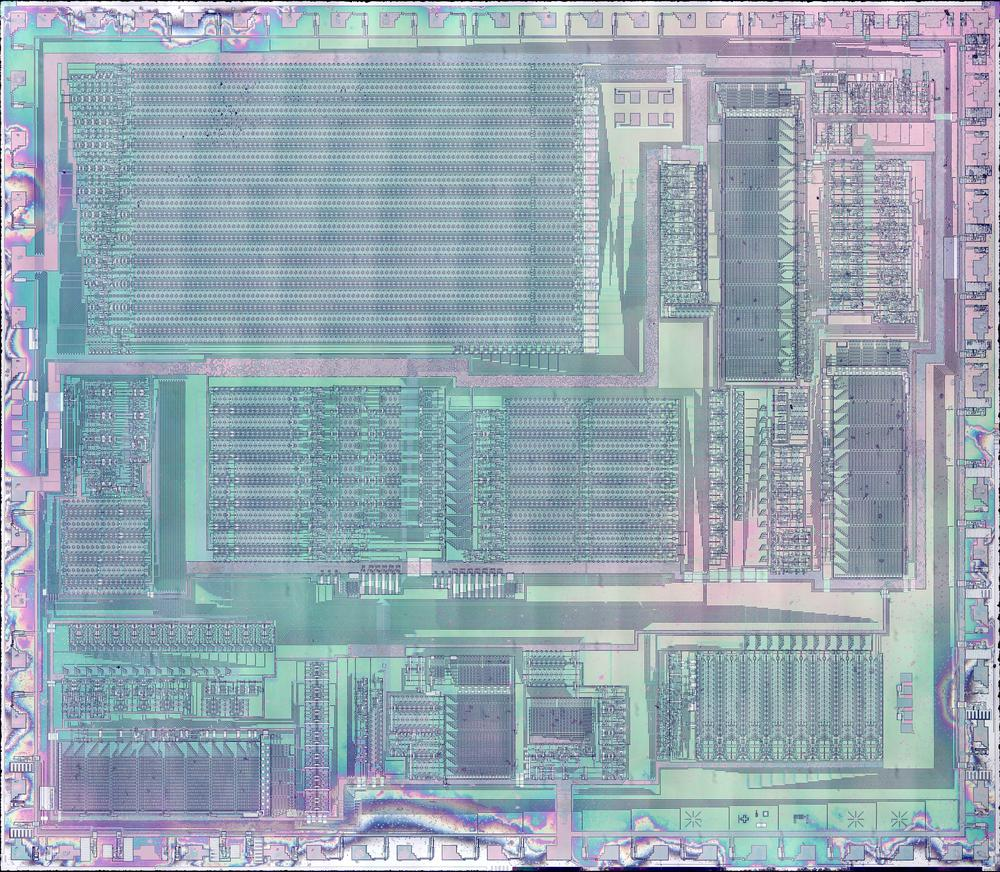Die photo of the DX7's YM21280 Operator chip. Click this photo (or any other) for a magnified version.

Instead of the analog oscillators and filters of an analog synthesizer, the DX7 generates sounds digitally, using a technique called FM synthesis. The idea is that you start with a sine wave (the carrier signal) and perturb it with another signal (the modulating signal). The modulating signal changes the phase (and thus the frequency) of the carrier, creating complex harmonic structures like the waveform below. These signals are represented as digital values throughout the system; a digital-to-analog converter (DAC) turns the digital representation into an analog voltage for the synthesizer's output.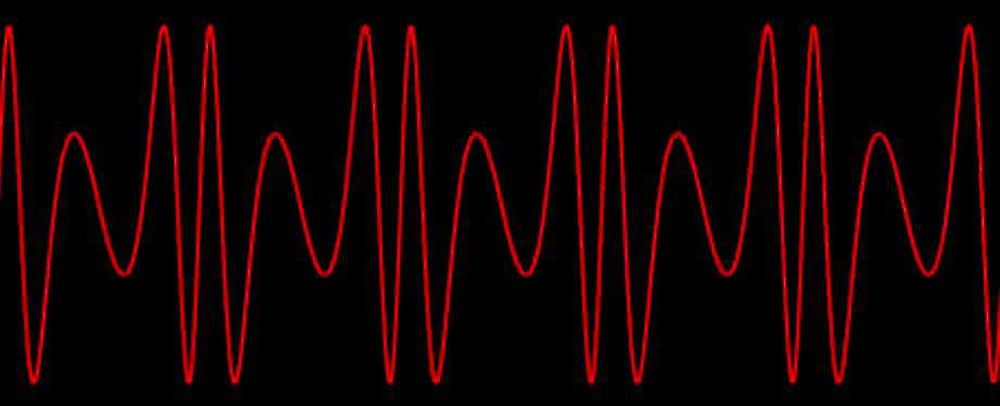An example of a complex waveform created by FM synthesis. (I made a tool that lets you experiment with FM synthesis.)

The digital implementation of frequency modulation uses a lookup table that holds a digitized sine wave. By stepping an index through the table at a specific rate, you can produce a sine wave of a fixed frequency. By perturbing this index with another signal, you can produce a modulated sine wave like the one below. The DX7 implements this with a sine-wave table in ROM, an increment value that controls the frequency, and an adder that adds the increment to the table index (i.e. the phase angle) each time step. This ROM is the subject of this blog post.

The amplitude of the sine wave is controlled by an envelope, varying over time; multiplying the sine wave by the envelope level yields the output. However, fast multiplication required too much hardware in the 1980s, so the DX7 uses a mathematical shortcut: adding logarithms is equivalent to multiplying the values. The obvious problem is that computing logarithms is harder than multiplying, but the trick is to store the (negated) logarithm of the sine wave in the lookup table (below) instead of the sine wave. This provides the logarithm for free. (The other issue is that you need to perform an exponential to get the final result. I described the exponential ROM and circuit in my previous DX7 article).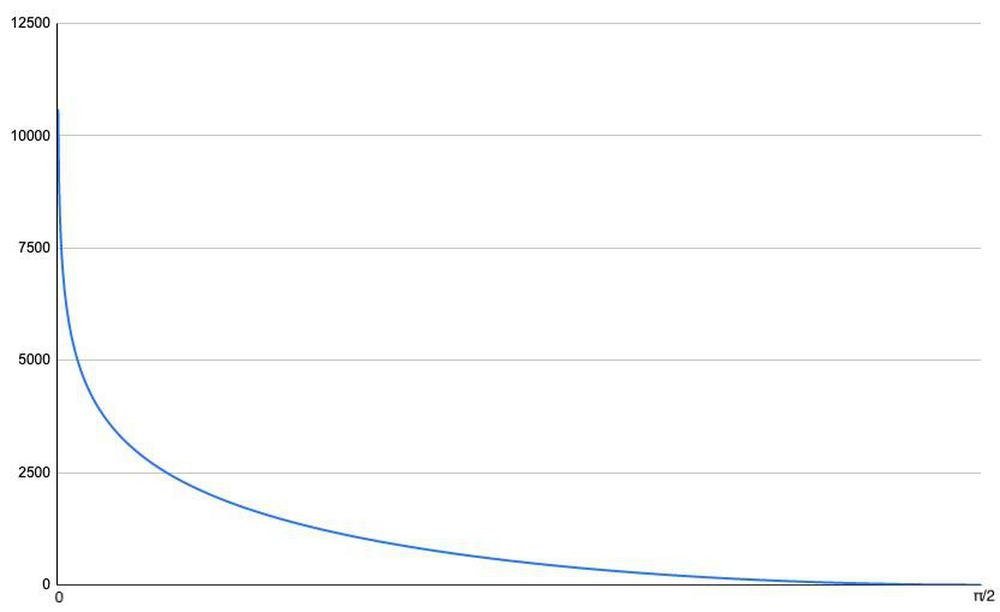This graph shows the log-sine function over one quarter of the wave, as a 14-bit value. It's not recognizable as a sine function, but will turn into a sine wave after exponentiation.

The block diagram below shows the structure of the log-sine circuit, computing a 14-bit value from a 12-bit input. The circuitry is somewhat complex to fit a fast, high-accuracy calculation into a small space on the die. The implementation takes advantage of the symmetry of the sine wave so only a quarter-wave needs to be stored. The top bit is used as the sign bit, which inverts the output elsewhere to obtain the negative half of the sine wave. (This also avoids the problem of taking the log of a negative value.) The second bit implements the mirror symmetry of each sine-wave peak by inverting the bits for the second half of the peak.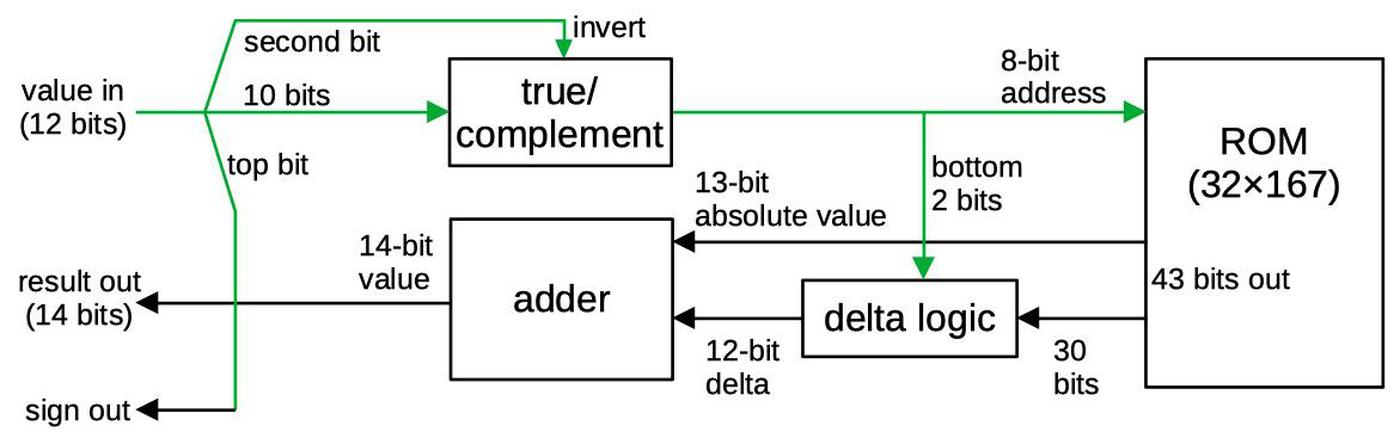Block diagram of the sine circuit. Input bits are indicated in green.

The ROM and associated logic take a 10-bit input address representing a quarter of the sine wave (angles 0 through π/2). A technique called delta encoding is used to reduce the size of the ROM. The idea of delta encoding is that if values change slowly, the difference between two values is considerably smaller than the value itself.1 Specifically, only every fourth value is explicitly stored in the ROM; this value is called an "absolute" value.3 The next three values are stored as deltas: the difference between the value and the previous absolute value.2 An adder circuit adds the absolute value to the difference value, yielding the desired log-sin value.

The diagram below labels the main functional blocks of the chip. In this article, I focus on the sine circuit, highlighted in red, but I'll summarize the other blocks. The 96 phase accumulators, implemented with shift registers, are the largest block of the chip. They hold the current table index for each of the DX7's 96 oscillators. The exponential function is implemented by two identical ROMs and associated addition/shifter circuitry. Other major blocks apply the envelope, hold configuration data, compute the operators that combine oscillators, define different operator algorithms, and buffer the output values.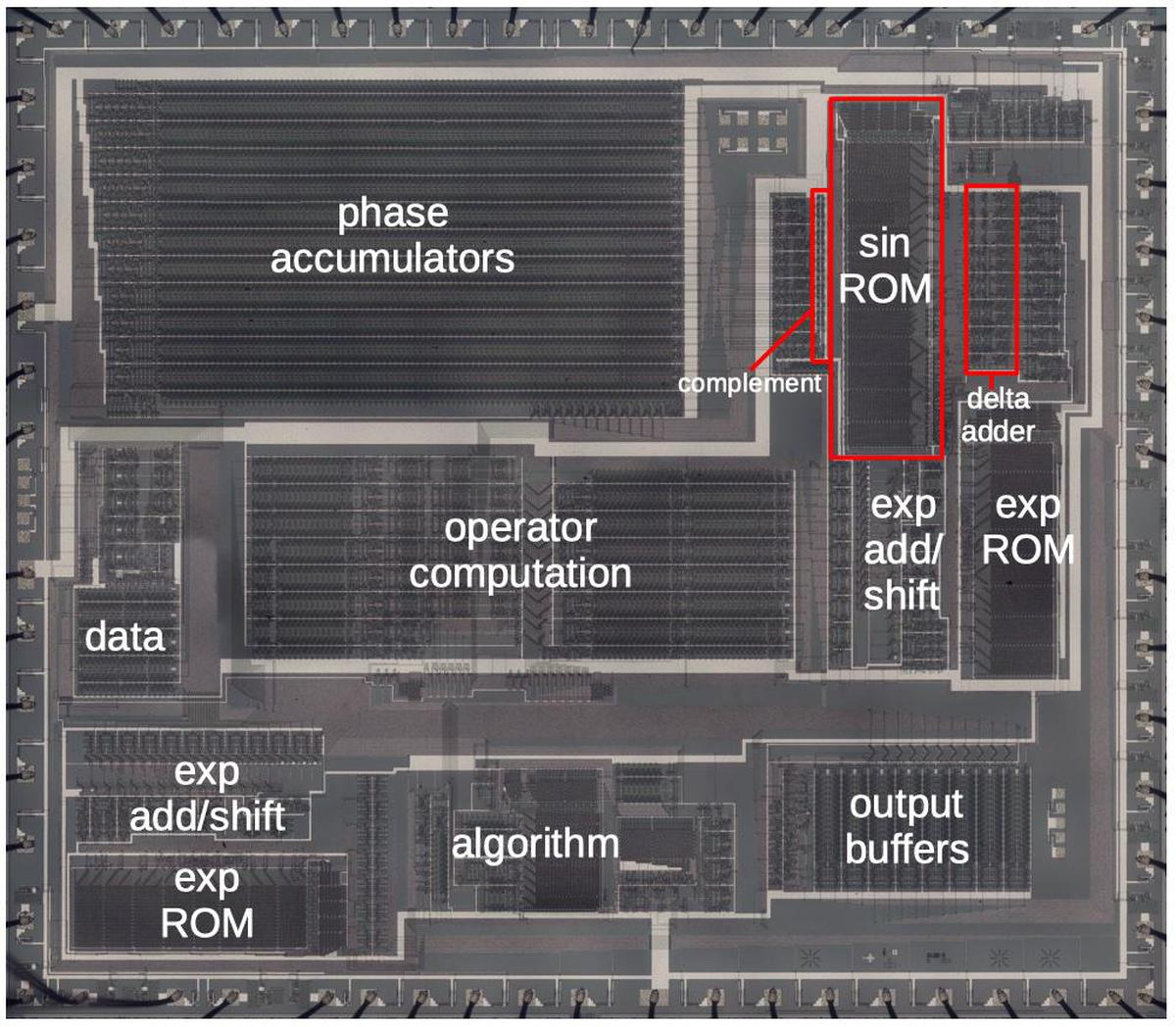Die with the major functional blocks labeled. This photo shows the metal layer of the chip. (Click for a larger version.)

## The ROM

The photo below shows the log-sin ROM. The ROM itself consists of a grid of transistors. At the top, decoder circuits select signal lines based on the address bits. At the right, the diagonal circuits are multiplexers, selecting particular rows of the ROM. To the right of the multiplexers, logic circuits select the delta values. I won't explain these circuits in detail since I discussed the similar circuits for the exponential ROMs in my previous article.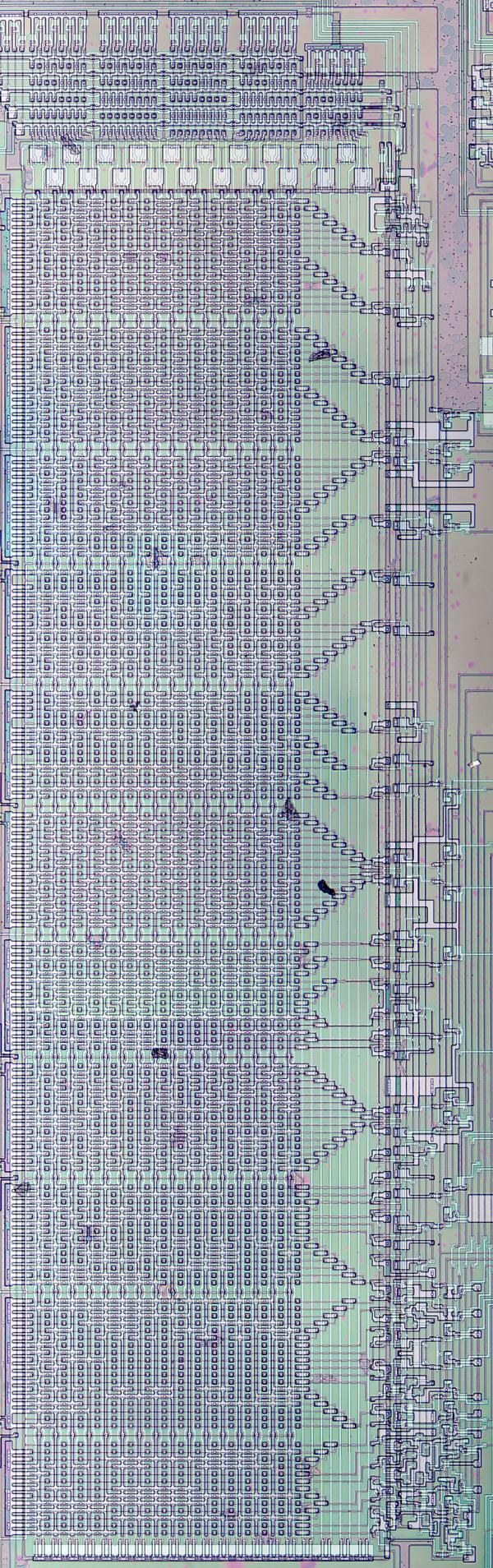High-resolution image of the sine ROM. Click this image (or any other) for an enlarged image.)

By examining the ROM closely, you can see the individual transistors that store bits. A transistor represents a 1, and the lack of a transistor represents a 0. Thus, the data in the ROM is created by the pattern of how the silicon is doped. I was able to read out the ROM data visually by looking at this pattern.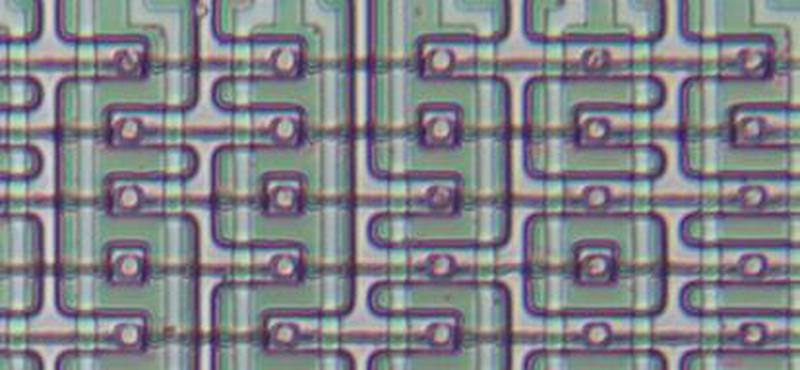Closeup of the ROM.

## The delta representation and the adder

The ROM itself produces 43 output bits, 13 bits for the "absolute" value and 30 bits for the three delta values. Some logic circuitry expands the ROM's 30 bits into three deltas of 12 bits (and a zero delta for the absolute value), taking advantage of some structure in the deltas. This circuitry is just to the right of the ROM and is implemented with AND-OR-INVERT gates. These gates implement 4-to-1 multiplexers, selecting the appropriate delta value based on the 2 lowest input bits.

Next, the adder circuit to the right of the ROM adds the 13-bit absolute value and the 12-bit delta value to generate the final 14-bit value. One interesting feature of the adder is it is pipelined to minimize the delay from carry propagation. I discussed the adder implementation in my previous article so I won't go into details here. The adder is immediately followed by a second adder that adds the envelope value to scale the signal level, taking advantage of the logarithmic representation.

Overall, the log-sine circuit generates 1024 14-bit values. Stored directly, this would take over 14 kilobits, but the ROM is only 5344 bits. The delta representation and ROM compression reduce the ROM size by almost 63%, important for a chip built in the 1980s when transistors were precious. By itself, the delta representation doesn't save much space: a 12-bit delta instead of a 14-bit value. But the ROM's implementation makes the deltas efficient: if a 32-bit row in the ROM is all zeroes, the row can be omitted entirely and the output defaults to 0. For the flat parts of the function, the high-order bits of the deltas are mostly zero, so much of the ROM can be omitted.

## Conclusion

The DX7 generates its waveforms from a digital sine wave, so producing a high-accuracy value rapidly is key to the synthesizer's performance. By examining the ROM and associated circuitry, I could obtain the exact values that the DX7 uses for the log-sine function. The ROM provides one quarter of the sine wave and the other quarters are formed by symmetry. For a 10-bit input value n, the corresponding angle is ω = (n + .5)/1024×π/24 and output value y is -log2(sin(ω)), represented as the integer round(y×1024).5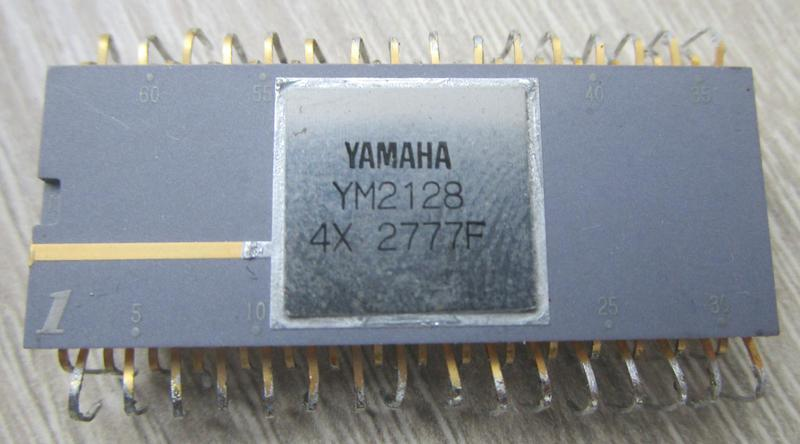The DX7's OPS chip comes in a 64-pin ceramic package with staggered pins. This is known as a Quad Inline Package (QIP). Photo courtesy of Jacques Mattheij.

Thanks to Jacques Mattheij and Anthony Richardson for providing the chip and discussion.6

## Notes and references

1. A different chip, the Yamaha YMF262 (1988) was used in computer sound cards such as the Sound Blaster 16. (This chip is also known as OPL3 for FM Operator Type-L.) It uses FM synthesis, but is stripped down compared to the DX-7. The chip was reverse-engineered by Matthew Gambrell and Olli Niemitalo who decapsulated the chip and read out the ROM contents.

The OPL3 log-sine ROM is similar to the DX7's in some ways, but is lower resolution. The OPL3 chip is 256 samples long, rather than 1024, and holds 8-bit values, rather than 13-bit values. Both chips use delta encoding, but the OPL3 has one delta-encoded value for each absolute value, while the DX7 has three delta-encoded values.

2. To be precise, the three delta values are stored before the absolute value in the ROM. That is, entries 3, 7, 11, ... are absolute, instead of 0, 4, 8, ..., the expected locations. I think this is because the log-sin function is decreasing, so if you want to add the deltas (instead of subtracting), the absolute value needs to be the last of the group, not the first.

3. The absolute value in delta encoding is the full, explicit value. It's unrelated to the absolute value function |x|.

4. Note that the input to the ROM is incremented by half a bit. This avoids duplication of the 0 value of the waveform when the quarter-wave is mirrored. It also avoids computation of the undefined value log(0).

5. The value is rounded to an integer by computing int(y×1024 + .5002). The constant .5002 rounds the value up, with just a tiny bit more that affects a single entry. I'm not sure why the rounding is not exact; perhaps Yamaha used a lower-precision sine or logarithm, which was just enough to change one bit. (Note that the value .0002 is somewhat arbitrary; a slightly larger or smaller number will yield the same result.)

6. For more information on the DX7 internals, see DX7 Technical Analysis, DX7 Hardware, OPLx decapsulated, and my previous DX7 articles Reverse-engineering the Yamaha DX7 synthesizer's sound chip from die photos and The Yamaha DX7's exponential circuit

### The Yamaha DX7 synthesizer's clever exponential circuit, reverse-engineered

The Yamaha DX7 digital synthesizer was released in 1983 and became extremely popular, defining the sound of 1980s pop music. Because microprocessors weren't fast enough in the early 1980s, the DX7 used two custom digital chips: the EGS "envelope" chip generated frequency and envelope data, which it fed to the OPS "operator" chip that generated the sound waveforms. A key part of the OPS chip is an exponential circuit, which is used for frequency calculation and envelope application. In this blog post, I examine this circuit—implemented by a ROM, shifter, and other circuitry—in detail and extract the ROM's data.

I created the high-resolution die photo below by compositing over a hundred microscope photos. Around the edges, you can see the 64 bond wires attached to pads; these connect the silicon die to the chip's 64 pins. The chip has one layer of metal, visible as the whitish lines on top. (Power and ground are the thick metal lines.) Underneath the metal, the polysilicon wiring layer appears reddish or greenish. Finally, the underlying silicon is grayish. I discussed the chip as a whole in my previous DX7 article; now I will focus on the exponential circuit.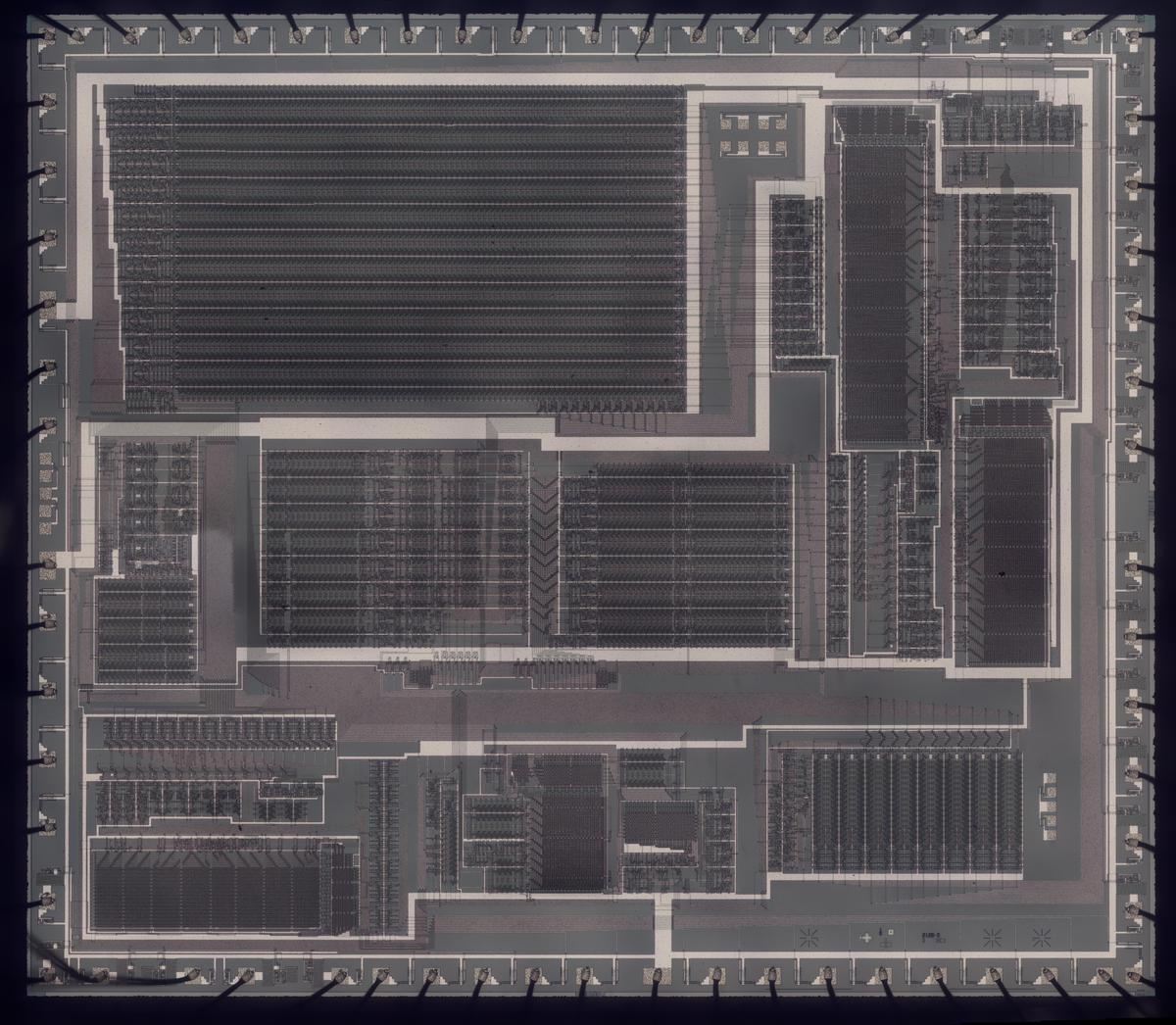Die photo of the DX7's YM21280 Operator chip. Click this photo (or any other) for a magnified version.

The DX7 was the first commercially successful digital synthesizer. Instead of the analog oscillators and filters of an analog synthesizer, the DX7 generates sounds digitally, using a technique called FM synthesis. The idea is that you start with a sine wave (the carrier signal) and perturb it with another signal (the modulating signal). The modulating signal changes the phase (and thus the frequency) of the carrier, creating complex harmonic structures. These signals are represented as digital values throughout the system; a digital-to-analog converter (DAC) turns the digital representation into an analog voltage for the synthesizer's output.

The digital implementation of frequency modulation uses a lookup table that holds a digitized sine wave. By stepping an index through the table at a specific rate, you can produce a sine wave of a fixed frequency. By perturbing this index with another signal, you can produce a modulated sine wave. The DX7 implements this with a sine-wave table in ROM, an increment value that controls the frequency, and an adder that adds the increment to the table index (i.e. the phase angle) each time step. The DX7 has 96 oscillators, so it keeps track of 96 separate phase angles; these are stored in the phase accumulators. The frequency modulation is implemented by operator circuitry, which allows oscillators to perturb other oscillators. (This is a very brief overview of FM synthesis; see my previous DX7 reverse-engineering article for more details.)

## Logarithms and exponentials

In hardware, multiplication is much slower than addition, especially with 1980s-era technology. The solution in the DX7 is to represent values as base-2 logarithms because adding logarithms is equivalent to multiplying the values. By applying 2x to the sum, the logarithmic value can be converted back to a linear value.

The first role for logarithms is in the frequency input to the chip: the phase increment value supplied to the chip is logarithmic. The motivation is that note frequencies are related exponentially: for instance, going up one octave doubles the frequency. By using logarithms, note computations can be done with addition.

Second, each oscillator has an associated envelope, which changes the output level according to a time-varying curve.1 To multiply the signal by the envelope level, the sine wave signal and the envelope are both represented logarithmically. Thus, the multiplication is replaced by addition. (The logarithm of the sine-wave signal is conveniently obtained by storing log2(sin(x)) in the waveform ROM instead of sin(x), so the logarithm is obtained "for free".)

The block diagram below shows the structure of the exponential circuit that converts the logarithmic value to a linear value by computing 2x. The exponential circuitry is somewhat complex to fit a fast, high-accuracy exponential calculation into a small space on the die. The circuit takes a 14-bit input value that consists of a 4-bit integer part and 10 fractional bits, so it computes 2x for 0≤x<16.2 The circuit uses a ROM lookup and a shift to rapidly compute the value.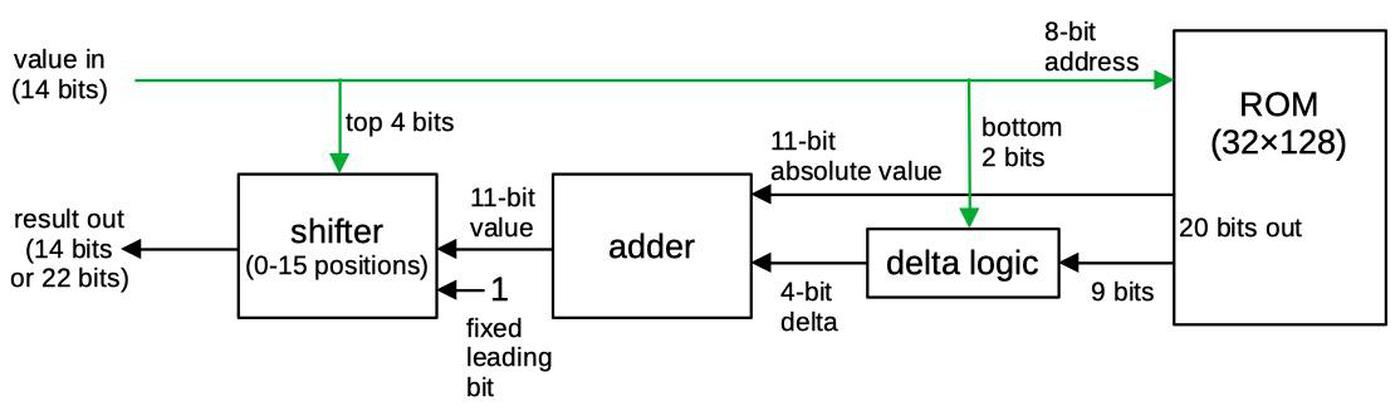Block diagram of the exponentiation circuit. Input bits are indicated in green.

The ROM takes a 10-bit input address (0 through 1023) representing x values 0 through 1023/1024. A technique called delta encoding is used to reduce the size of the ROM. The idea of delta encoding is that if values change slowly, the difference between two values is considerably smaller than the value itself.3 Specifically, only every fourth value is explicitly stored in the ROM; this value is called an "absolute" value.7 The next three values are stored as the deltas, difference between the value and the previous absolute value. The deltas fit into 4 bits4, a considerable saving over the 11-bit absolute values. An adder circuit adds the absolute value to the difference value, yielding the desired exponential value.

The final step in the exponential circuity is to perform a binary shift on the value from the ROM. Shifting by the number of bits in the integer part of the input results in the final exponential value.6 Prior to shifting, a leading 1 is added to the ROM's value so this bit doesn't take up space in the ROM.5 The chip has two exponentiation circuits: one for computing the frequency and one for computing the signal (the sine and envelope path). Most of the circuitry is identical between the two, but the frequency exponent produces 22 bits of output, while the signal exponent has just 14 bits of output.

## A closer look at the die

The diagram below labels the pins and the main functional blocks of the chip. In this article, I focus on the two exponential circuits, highlighted in red, but I'll summarize the other blocks. The 96 phase accumulators, implemented with shift registers, are the largest block of the chip. ROMs hold the sine wave function and the exponential function. (There are two identical exponential ROMs, with associated adder and shifter circuitry.) Other major blocks apply the envelope, hold configuration data, compute the operators that combine oscillators, define different operator algorithms, and buffer the output values.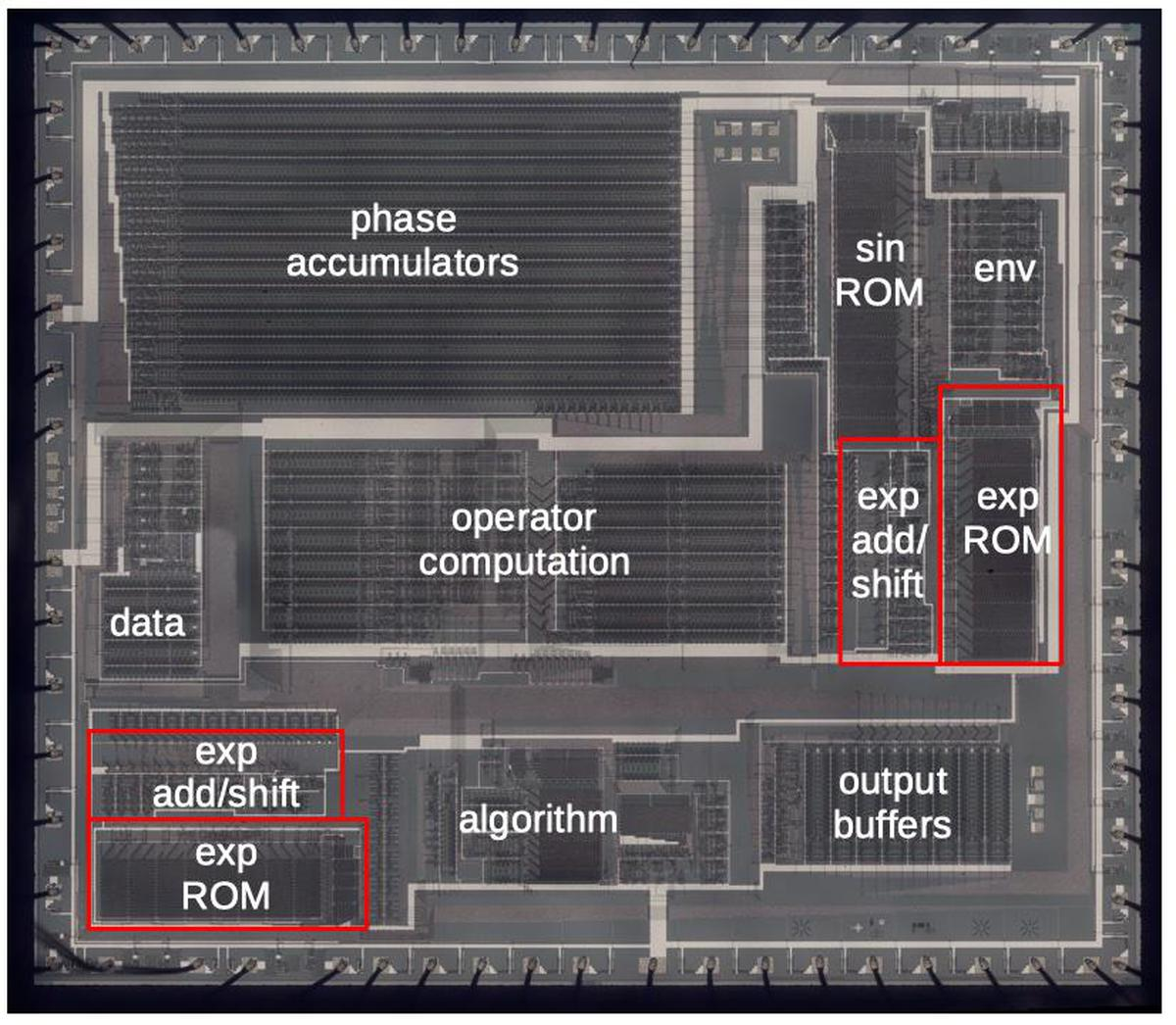Die with the major functional blocks labeled. This photo shows the metal layer of the chip. (Click for a larger version.)

### Transistors

To explain the die photos, I'll first show how a transistor (below) is constructed in an NMOS integrated circuit. Regions of the silicon are doped with impurities to create diffusion regions with desired properties. The transistor can be viewed as a switch, allowing current to flow between two diffusion regions called the source and drain. The transistor is controlled by the gate, made of a special type of silicon called polysilicon. A high voltage on the gate lets current flow between the source and drain, while a low voltage blocks current flow. These tiny transistors are combined to form logic gates and other circuits.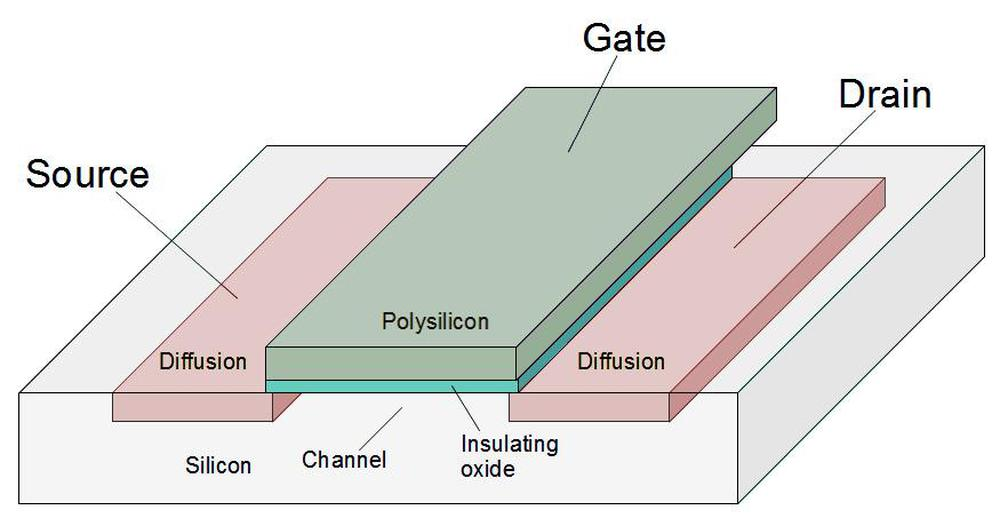Structure of an NMOS transistor (MOSFET) as implemented in an integrated circuit.

To make the transistors more visible, I removed the metal layer from the chip, resulting in the high-resolution die photo below. (The colors are due to variations in the thickness of the oxide layer due to my etching process.)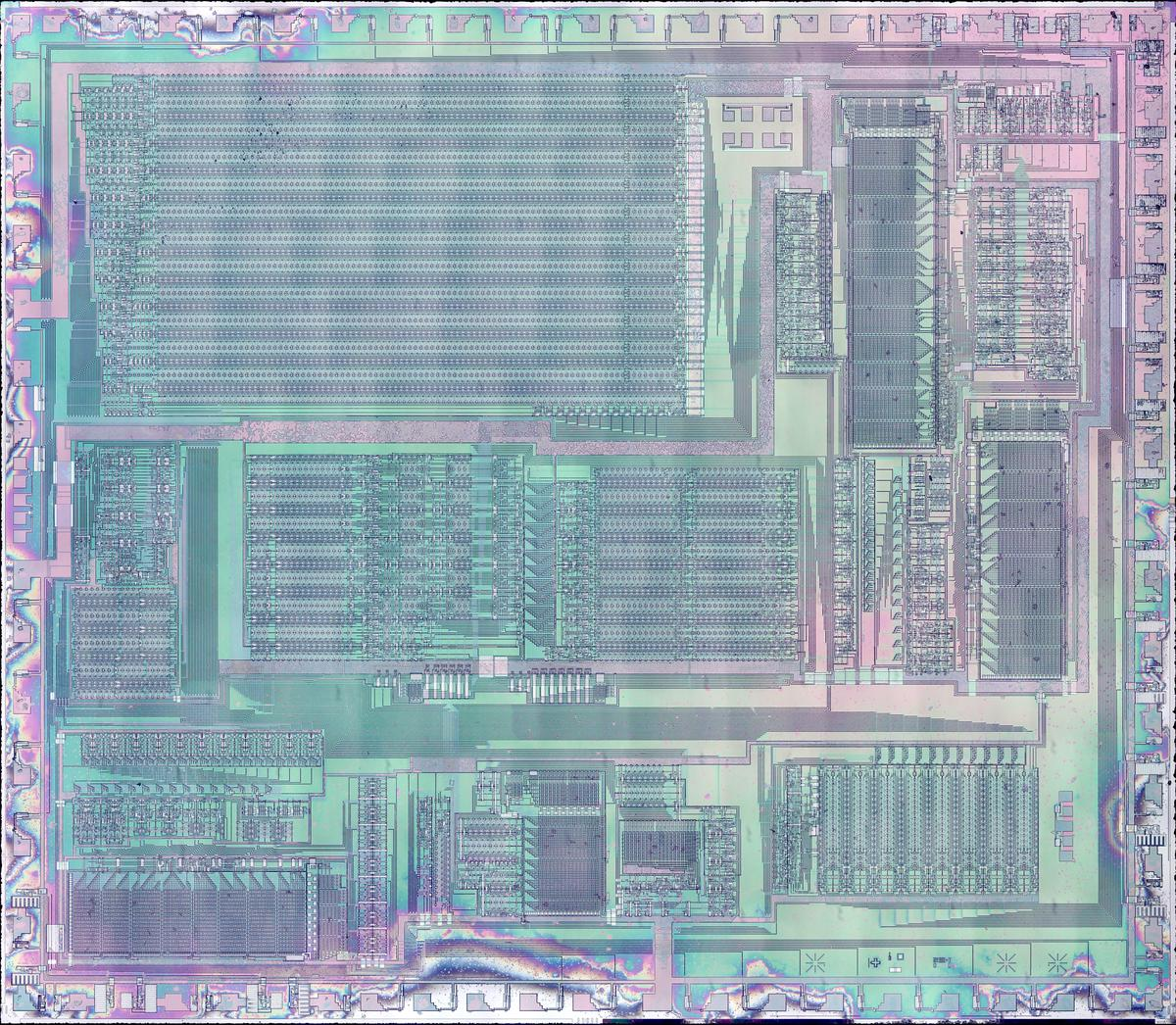Die photo of the DX7's YM21280 Operator chip with the metal layer removed. Click this photo (or any other) for a magnified version.

### The ROM

The diagram below shows one of the exponential ROMs. The ROM is constructed from a grid of transistors: 128 rows by 32 columns.8 At each grid point, a transistor can be present, representing a 1 bit; or a transistor can be absent, representing a 0 bit.9

At the top, decoders activate one of the 32 vertical select lines in the ROM, based on five bits of the address. The ROM is arranged into groups of 8 rows (or fewer, depending on compression). A multiplexer selects one bit of each group, based on three bits of the address. This produces a 20-bit output. Finally, the output logic produces the desired delta value, based on the address. The result is the 11-bit absolute value and a 4-bit delta value.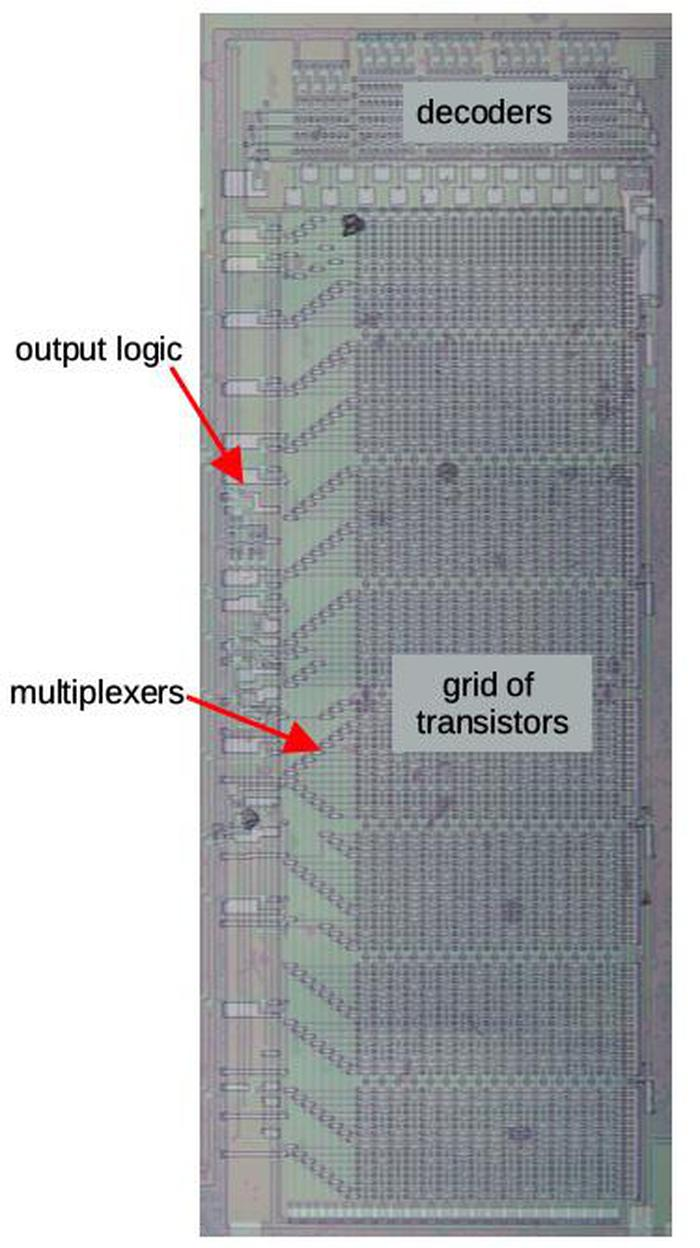The ROM with the main components labeled.

Zooming in on the ROM shows the individual transistors. The large pale regions are the doped silicon, forming transistor sources and drains. The polysilicon select lines are vertical. A transistor is formed when a polysilicon line crosses a doped silicon region. The indicated silicon regions are connected to ground, pulling one side of each transistor low. The circles are connections called vias between the silicon and the metal lines above. (The metal lines have been removed but the wavy horizontal lines show where the metal was.)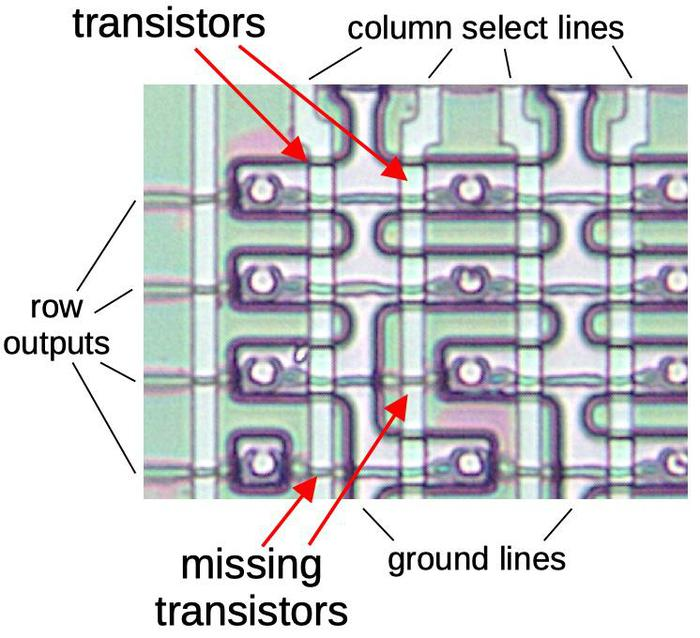Closeup of a 4×4 section of the ROM, showing its construction.

Each bit is stored in the ROM by the presence or absence of a transistor at a grid position. (During manufacturing, the silicon doping pattern controls whether or not a transistor exists.) When one of the 32 select lines is activated, all the transistors in that column will turn on, pulling the corresponding output lines low. But if a transistor is missing, the corresponding output line will remain high. Thus, a value is read from the ROM by activating a select line, reading that ROM value onto the output lines. By looking at the silicon pattern in the ROM, I determined the sequence of 1's and 0's stored in the ROM, 4 kilobits in total.

### The multiplexer

The ROM has 256 entries of 20 bits, before the delta processing is applied. To make the layout more efficient, the ROM stores bits in groups of 8, (conceptually) organized as 8 rows of 32 entries (columns) for each output bit. Each output bit has a multiplexer that selects one of the 8 bits in the group, based on 3 more address lines that control the 8 multiplexer select lines.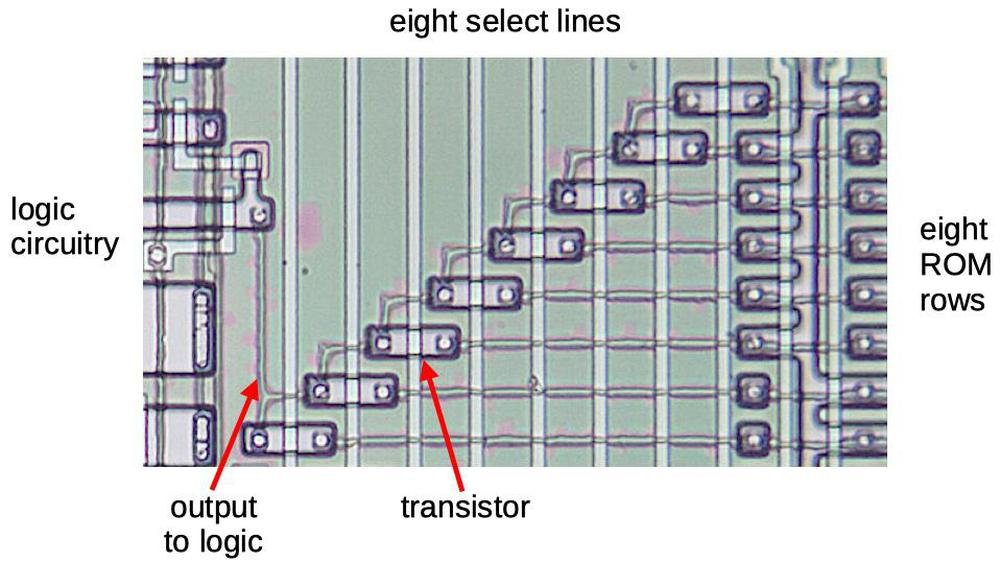A multiplexer in the ROM.

Each multiplexer (above) is implemented by 8 pass transistors. One transistor is activated, letting that row's bit through, while the unselected rows are blocked. The output of the multiplexer goes to the logic circuitry on the left.

Looking at the die photo closely shows that some of the multiplexers don't have all eight rows. This is a key optimization to reduce the ROM size. If all the bits in a row are 0, the row can be eliminated from the ROM entirely.10

### The delta logic and the adder

The ROM produces 20 output bits, 11 bits for the "absolute" value and 9 bits for the three delta values. Some logic circuitry expands the ROM's 9 bits into three deltas of four bits, taking advantage of some structure in the deltas.11

To obtain the 2x-1 value, the 11-bit absolute value and the 4-bit delta must be added. This is accomplished by an adder circuit to the left of the ROM. One interesting feature of the adder is it is pipelined to minimize the delay from carry propagation. I discussed the adder in my previous article so I won't go into details here.

### The bit shifter

The final building block that I'll discuss is the bit shifter, which implements the integer part of the exponential calculation. It shifts the value to the left by 0 to 15 bits, which is equivalent to multiplying by a power of 2. The shifter is built in two layers: the bottom layer shifts by 0, 1, 2, or 3 positions. The upper layer shifts by 0, 4, 8, or 12 positions. The combination of the two layers permits any shift between 0 and 15 bit positions. Wiring between the two layers distributes the outputs from the first layer to the second layer. Each output goes to four inputs, each spaced 4 bits apart to provide the larger shift.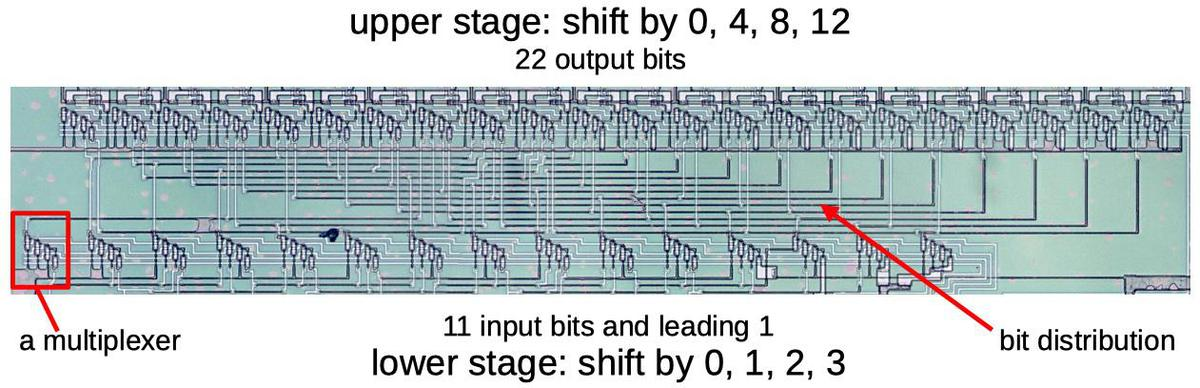The shifter circuit.

The diagram below shows part of the shifter that shifts by 0, 1, 2, or 3 positions, controlled by the horizontal lines. The shifter is built from multiplexers, similar to those in the ROM, that select one of four inputs. I've highlighted one of the bits in green. If the "shift 0" line is activated, the rightmost green transistor (circled) will turn on and the green input bit will exit from the rightmost output. Likewise, if the "shift 1" line is activated, the second green transistor will turn on and the green bit will exit shifted one position to the left. The "shift 2" and "shift 3" lines will cause the green bit to be shifted two or three positions to the left. The remaining transistors (circled in black) act in the same manner to shift the other bits. The result is that all the bits will be shifted by shifted 0, 1, 2, or 3 positions. The second shifter is similar, except the input lines go to multiplexers that are four positions apart.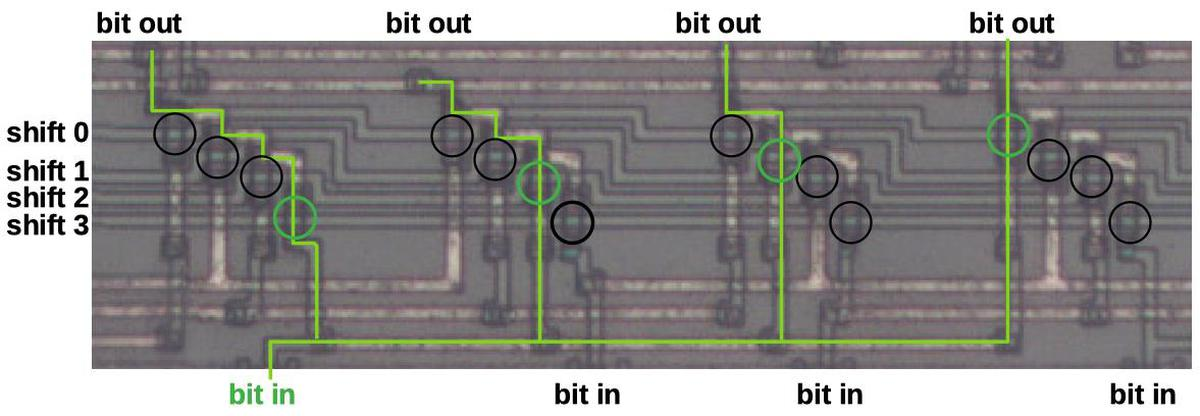Detail of the shifter circuit.

## Conclusion

Computing exponents is a key part of the DX7's sound synthesis. The chip needs to compute exponents very quickly, faster than an algorithm such as CORDIC could operate, but a straightforward ROM would have been much too large. The chip solves this dilemma by using delta encoding, ROM compression, and a shifter circuit. These techniques reduced the ROM size by almost 64%.12 By examining the circuitry closely, I have reverse-engineered the exact values that are generated. DX7 emulators may be able to achieve more accuracy by using these values.The DX7's OPS chip comes in a 64-pin ceramic package with staggered pins. This is known as a Quad Inline Package (QIP).

The next step is to reverse-engineer the chip's sine wave ROM, which implements the log-sin function. That ROM uses many of the same techniques as the exponential ROM, but stores the deltas differently, for instance. I announce my latest blog posts on Twitter, so follow me @kenshirriff for updates. I also have an RSS feed. If you're interested in ROM data, I also wrote about extracting constants from the 8087 floating point chip.

Thanks to Jacques Mattheij and Anthony Richardson for providing the chip and discussion.14

## Notes and references

1. For an output signal, the envelope gives the note a more realistic sound; a typical sound has a sharp attack when it is first played, and then the volume decays. The level is sustained until the key is released, and then dies off quickly. However, the DX7 also applies envelopes to the modulating signals, allowing the timbre of the note to change over time.

2. The exact values of the exponentiation circuit are given as follows. Suppose the 14-bit input value is int.frac, where int is the 4-bit integer part and frac is the 10-bit fractional part. The 12-bit output value from the ROM, after the delta adder and appending a leading 1 bit, is exactly given by round(2frac×2048). The shifter applies a left shift of 0 to 15 bits and then the result is truncated to 22 or 14 bits, for the frequency and signal exponentiation respectively. The final results are
round(2frac× 2048) << int >> 5, and
round(2frac× 2048) << int >> 13, respectively
(where << and >> are the bit shift operators).

In both cases, the fixed 1 is in the leftmost position of the output when the input has maximum integer portion (i.e. 15). (This is necessary since otherwise the value would either get truncated, or the leftmost bit would be unused.) However, with input integer portion of 0, the frequency circuit still has 7 bits of output, while the envelope circuit produces a value of 0 (since all the bits are lost in shifting).

3. A different chip, the Yamaha YMF262 was used in computer sound cards such as the Sound Blaster 16. (This chip is also known as OPL3 for FM Operator Type-L.) It uses FM synthesis, but is stripped down compared to the DX-7. The chip was reverse-engineered by Matthew Gambrell and Olli Niemitalo who decapsulated the chip and read out the ROM contents.

The OPL3 exponential ROM is similar to the DX7's in some ways, but is also very different. The OPL3 chip is 256 samples long, rather than 1024, and holds 10-bit values, rather than 12-bit values. Both chips use delta encoding, but the OPL3 has one delta-encoded value for each absolute value, while the DX7 has three delta-encoded values.

4. The graph below shows the exponential function 2x over the fractional range. The difference between successive elements is fairly small, so a 4-bit delta value is sufficient. Storing a 4-bit difference instead of an 11-bit absolute value achieves a large space saving.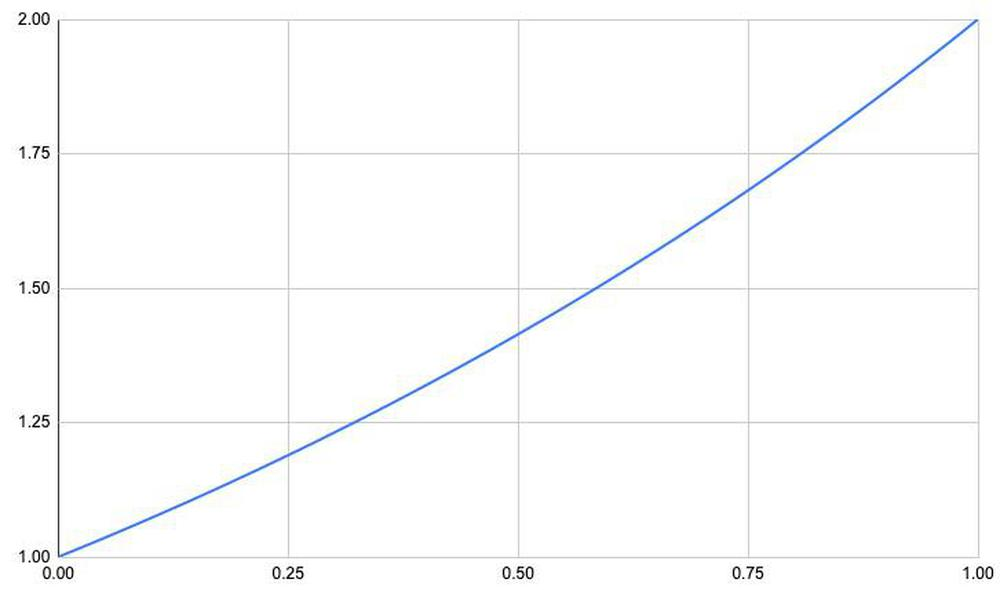Graph of 2x over the fractional range.

Since the exponential function is convex, the largest delta in the exponential table is at the right, specifically (21023/1024-21020/1024)×2048 ≈ 8.3. The delta almost fits into three bits, but four bits are required.

5. The ROM stores 2x-1 rather than 2x, since all the values have a leading one. Specifically, for 0≤x<1, 1≤2x<2. Adding 1 to the ROM's output instead of explicitly storing it in the ROM reduces the ROM's size.

6. Mathematically, if the input value is split into integer and fractional parts: int+frac, then 2int+frac = 2int×2frac. Multiplying by 2int is the same as performing a binary shift int bits to the left.

7. The absolute value in delta encoding is the full, explicit value. It's unrelated to the absolute value function |x|.

8. The two exponential ROMs on the chip are identical, except one is horizontal and one is vertical. This makes referring to rows and columns a bit ambiguous; hopefully it all makes sense.

9. Whether a transistor in the ROM represents a 1 or a 0 is somewhat arbitrary, since the signal gets inverted several times before use. A transistor will cause that line of the ROM to be pulled low, so at the fundamental level a transistor represents a 0. However, in the exponential ROM, this value is immediately inverted, so a transistor represents a 1 bit in the final result.

10. The ROM operates in two phases, controlled by the clock. In the first phase, the rows and the multiplexers are all pulled high. In the second phase, the desired ROM column is activated. If there are transistors, they will pull the rows low. Through the selected multiplexer transistor, this will pull the multiplexer low. The multiplexer output is then inverted, so a position with a transistor represents a logical 1 and the absence of a transistor represents a logical 0. With this circuit, if a row and multiplexer transistor are omitted entirely, the multiplexer will retain its high precharge value, which represents a logical 0. Thus, any rows in the ROM that are all 0 can be eliminated, saving space.

11. The schematic below shows the implementation of the logic to produce the absolute data and the deltas. The 11 absolute data bits simply take the corresponding multiplexer output and invert it. Each multiplexer also has a transistor to precharge it to +5 on the clock phase 1. (The delta multiplexers also have precharge transistors, but I omitted them from the schematic to avoid clutter.)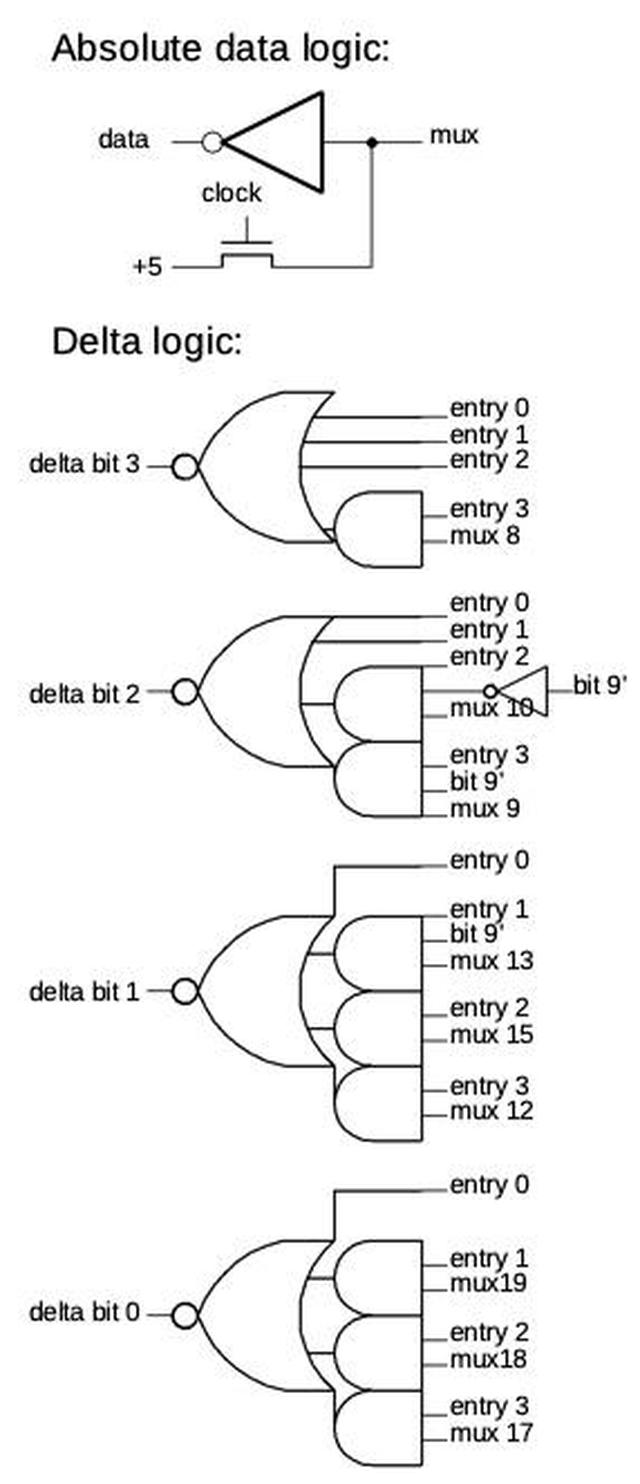Diagram of the logic circuitry.

The delta bit logic implements four different cases. Entry 0 provides a delta value of 0 for the absolute value. It is followed by three entries for the values stored as deltas. (In all four cases, the value is computed by adding the absolute value and the delta.) The two low-order address bits select the entry. If the 9 bits from the ROM are labeled A-I, the successive 4-bit delta entries are 0000 (no delta for the "absolute" value), 00AB, 0CDE, FGHI. Three entries use the top bit of the address (bit 9) to force a delta bit to 1 over half the range. This is another optimization so those regions don't need to be stored in the ROM.

12. The exponential circuit takes a 14-bit input and produces a 22-bit output. Holding all these values in a ROM would take over 360 kilobits, impractical in the 1980s. The use of a shifter dramatically reduced the storage requirement to 1024 11-bit values (11 Kb). The ROM compression techniques reduced this to just 4 kilobits, almost 64% less. In this section I break down how the ROM compression is implemented.

The majority of the savings comes from the delta encoding, which uses 256 11-bit "absolute" values and 768 4-bit delta values. This reduces the storage to about 5.9 Kb, saving about 48%. The remainder of the savings comes from eliminating rows in the ROM through various techniques. The ROM is structured as rows of 32 bits. Uncompressed, the ROM would require 184 rows. However, if the values in a row are all 0, the row can be omitted entirely, due to the multiplexer's construction.13 Since the exponential curve grows slowly, the top bits of the absolute value are 0 for large stretches, so many rows can be eliminated. Specifically, the topmost bit is zero for 4 of 8 rows, the next bit zero for 3 of 8 rows, and the next bit zero for 1 row. Thus, 8 rows can be eliminated for the absolute value storage.

The delta bits are also zero much of the time. The top two bits of the first delta are always 0, as is the top bit of the second delta. This is handled by the logic circuitry, eliminating 24 rows of the ROM. 12 more zero rows are eliminated from delta bits that are zero some of the time. Finally, the logic circuitry forces 3 delta bits to 1 over half-intervals where they are always 1, making 12 more rows unnecessary.

To summarize, zero-row-elimination saves 8 rows from absolute value data, and 36 rows from delta data. Another 12 rows are saved by forcing bits to 1. This reduces the ROM from potentially 188 rows to the 128 rows it has, shrinking it almost 32%.

13. Conceptually, rows in the ROM can be considered NOR gates with pull-up resistors. However, the implementation is slightly different: rows are precharged to +5 during one clock phase and then discharged (or not) to ground through transistors. This reduces the power consumption compared to regular NMOS pull-ups. (Modern circuits use CMOS instead of NMOS to avoid the static power consumption of pull-ups.)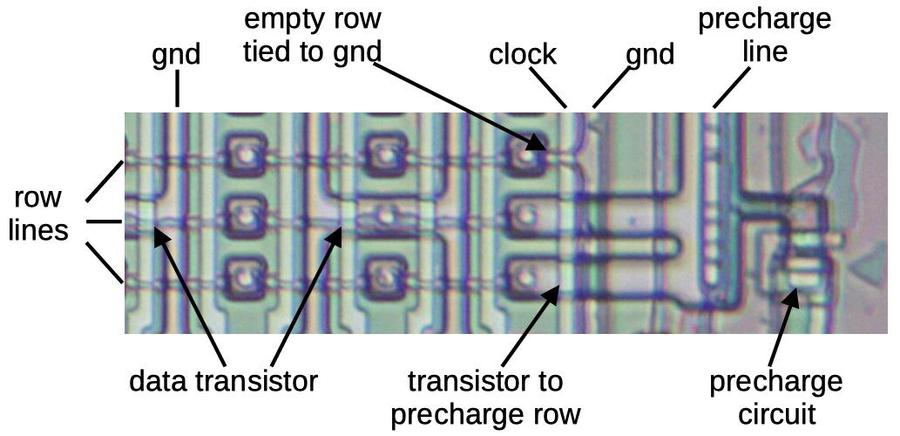The ROM with its precharge circuit. This is a bit tricky to interpret. The row lines are metal as is the ground line on the right. The other ground lines and the precharge line are in silicon. The clock and the column select lines (unlabeled) are in polysilicon.

Another optimization is that rows in the ROM that are all 1's have the transistors omitted and the output line connected directly to ground. This reduces power consumption slightly, since that row line won't be charged and discharged. However, it doesn't save any space, since the row is still physically present. (In contrast rows that are all 0's are omitted entirely.)

14. For more information on the DX7 internals, see DX7 Technical Analysis, DX7 Hardware, OPLx decapsulated, and my previous DX7 article Reverse-engineering the Yamaha DX7 synthesizer's sound chip from die photos

### Reverse-engineering the Yamaha DX7 synthesizer's sound chip from die photos

The Yamaha DX7 digital synthesizer was released in 1983 and became "one of the most important advances in the history of modern popular music"1. It defined the sound of 1980s pop music, used by bands from A-ha and Michael Jackson to Dolly Parton and Whitney Houston. The DX7's electric piano sound can be heard in over 40% of 1986's top hits.2 Compared to earlier synthesizers, the DX7 was compact, inexpensive, easy to use, and provided a new soundscape.3

While digital synthesis is straightforward nowadays, microprocessors4 weren't fast enough to do this in the early 1980s. Instead, the DX7 used two custom chips: the YM21290 EGS "envelope" chip generated frequency and envelope data, which it fed to the YM212805 OPS "operator" chip that generated the sound waveforms. In this blog post, I investigate the operator chip and how it digitally produced sounds using a technique called FM synthesis.6 21

I created the high-resolution die photo below by compositing over a hundred microscope photos.6 Around the edges, you can see the 64 bond wires attached to pads; these connect the silicon die to the chip's 64 pins. The chip has one layer of metal, visible as the whitish lines on top. (Power and ground are the thick metal lines.) Underneath the metal, the polysilicon wiring layer appears reddish or greenish. Finally, the underlying silicon is grayish. The overall layout of the chip is dense rectangles of circuitry with the space between them used for signal routing. I will discuss these circuitry blocks in detail below.Die photo of the DX7's YM21280 Operator chip. Click this photo (or any other) for a magnified version.

The photo below shows the integrated circuit with the metal lid removed, showing the silicon die inside. The pins have been flattened in the photo; they are normally bent downwards, but in a staggered pattern.7 The four rows of pins make this a quad in-line package, with twice the pin density as a regular DIP chip. As a result, this 64-pin chip has a smaller package than a standard 40-pin DIP chip.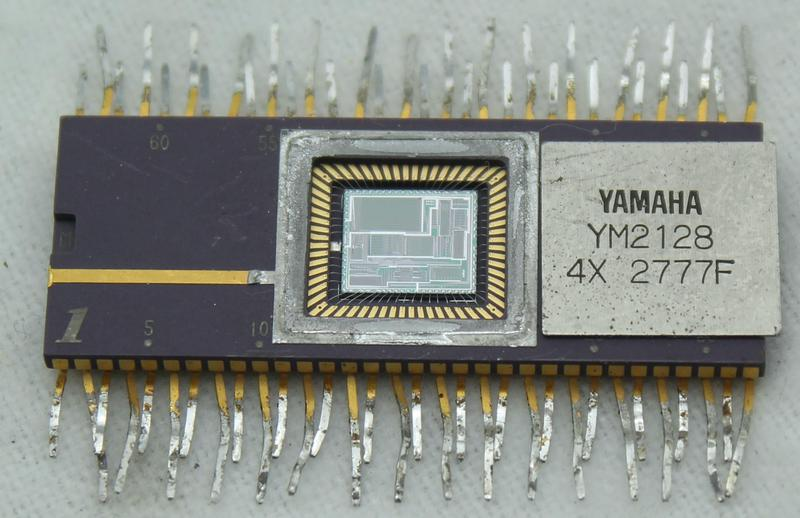The integrated circuit package with the metal lid removed, revealing the silicon die. Pin numbers are printed on the package, which is unusual.

## Analog and digital

In the 1960s and 1970s, synthesizers were mostly analog.8 An oscillator was controlled by the keyboard, generating a wave at the appropriate frequency. This signal was fed through a filter, which shaped the frequency spectrum to produce the desired tone quality (timbre). Finally, the signal had its volume shaped by an envelope generator that made the volume ramp up when the key was pressed, and die off gradually when the key was released.9

An analog synthesizer was built from components such as resistors, capacitors, and op-amps, with analog voltages as the signals. One problem was that the analog synthesizers needed to be tuned since these component values could drift over time. Another problem was that the complex circuitry generated one note, so analog synthesizers were typically monophonic, producing a single note at a time. The functions of an analog synthesizer were typically controlled by patch cords, potentiometer knobs, and switches, which allowed a wide variety of sounds to be produced. This made it difficult to select the desired sound, since all the parameters needed to be set manually.

Digital synthesis provided a completely different way of generating sounds. The sound values were produced digitally by an algorithm that generated numeric values. These values were converted to the output signal voltages by a digital-to-analog converter (DAC). Digital synthesizers solved many of the problems of analog synthesis: they could easily play multiple notes at once (i.e. polyphony), configurations could be stored as digital files, they could be controlled digitally10, they replaced precision analog components with cheaper digital circuits, and they produced new classes of sounds. The DX7 wasn't the first digital synthesizer, but it was the first to achieve commercial success. It became one of the best-selling synthesizers ever, with over 150,000 sold.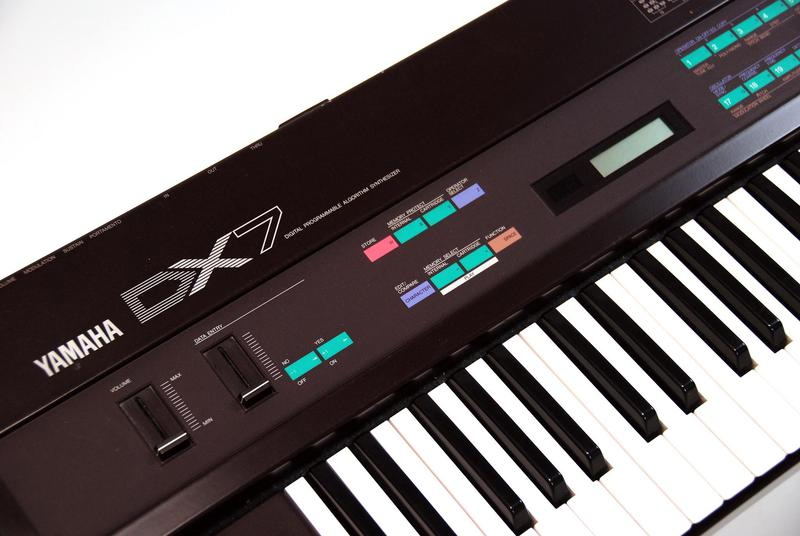The Yamaha DX7 synthesizer with its 61-key keyboard and digital controls. Photo by rockheim (CC BY-NC-SA 2.0).

## FM synthesis

The DX7 uses FM synthesis to generate its sounds.11 The idea is that you start with a sine wave (the carrier signal) and perturb it with another signal (the modulating signal). The modulating signal changes the phase (and thus the frequency) of the carrier, creating complex harmonic structures.

The digital implementation of frequency modulation starts with a lookup table that holds a digitized sine wave. By stepping an index through the table at a specific rate, you can produce a sine wave of a fixed frequency. To make this concrete, suppose the table is 4096 entries long and the index is updated at 40960 Hertz. If you increment the index by 100 each time, you'll cycle through the table 1000 times every second, so a sine wave at 1 kHz will be produced. The index represents the phase of the signal: as the index moves through the table, this corresponds to a phase of 0 to 2π and an output of sin(0) through sin(2π). Changing the increment value controls the frequency. For instance, an increment of 44 would produce 440 Hz.12

The next step is to modulate the output by adding a modulation signal to the index. When the modulation signal increases, the index will move through the table faster, increasing the output frequency. When the modulation signal decreases, the index will step through more slowly, decreasing the output frequency.

Digital synthesis can be implemented with straightforward hardware: a sine-wave table, an increment value that controls the frequency, and an adder that adds the increment to the table index (phase angle) each time step. Frequency modulation can be implemented by another adder to add the modulation value to the table index (phase angle).

The interactive tool below illustrates FM synthesis and the effects of changing the modulation frequency and amount of modulation.13 The modulation signal is shown in yellow and the output is shown in red. (The carrier is fixed at 440 Hz.) Low levels of modulation distort the output waveform, while high levels create very complex waveforms. If the modulation and carrier frequencies have integer ratios, the output is periodic. But a detuned modulation frequency results in a complex, more bell-like sound.

Modulation level: 1
Modulation frequency ratio: 2

As you can see, a single modulator produces a variety of timbres and complex, unpredictable waveforms. However, the DX7 provides multiple modulators combined in various ways, making the sounds vastly more varied. For each note, the DX7 provides six oscillators (called operators) that can be combined in 32 different ways (called algorithms), shown below. For example, in algorithm 1, operator 6 modulates operator 5 which modulates operator 4 which modulates operator 3, which produces a sound. Meanwhile, operator 2 modulates operator 1, producing a second sound. Other algorithms combine the six operators in different ways. The level of each operator is controlled by a different envelope, so the note's timbre can evolve in complex ways over time.14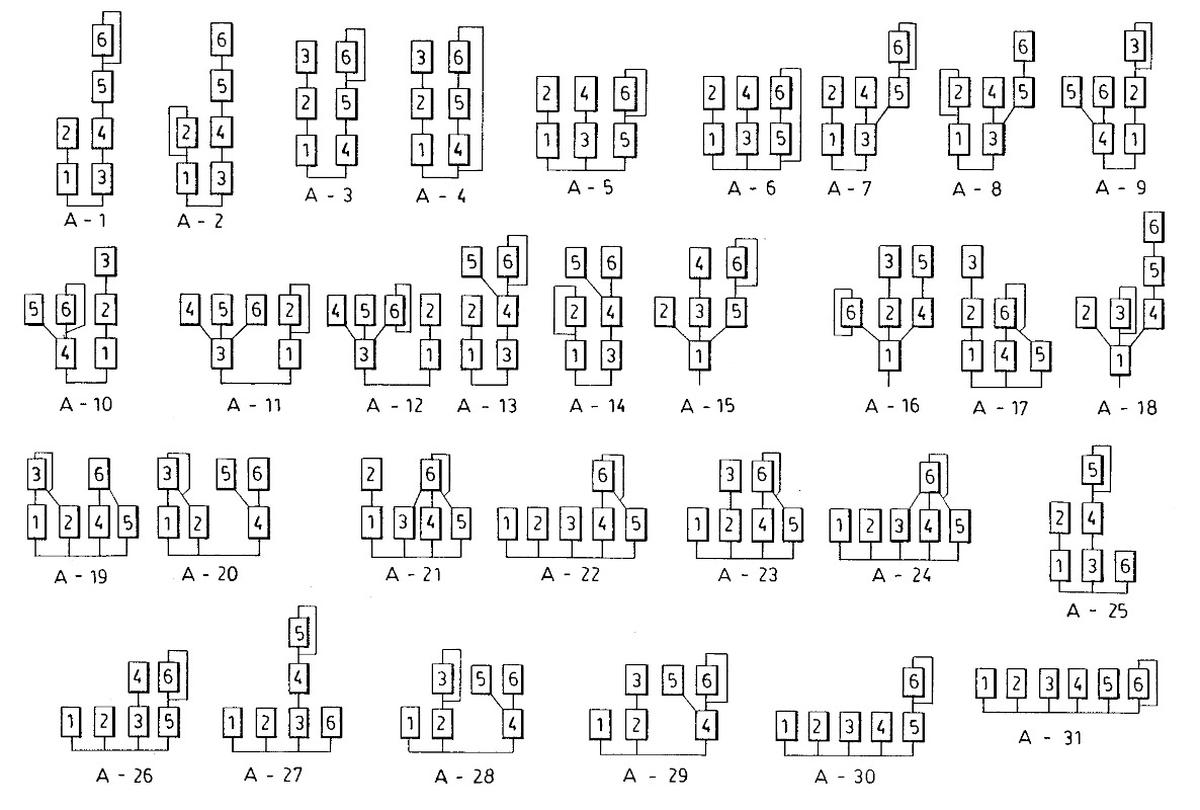A chart of the DX7's algorithms, from the patent.

## Inside the DX7

The DX7 can play 16 notes at once and each note has 6 operators, so there are 96 oscillators/operators in total. However, the circuitry operates sequentially, updating one oscillator and computing one operator at a time. The DX7 stores the current index (phase) values for each of the 96 oscillators but shares the circuitry that uses these values. Instead of RAM, the DX7 uses shift registers to hold data, in particular 96-stage shift registers to hold the 96 phase values. This approach drastically reduces the hardware requirements compared to using 96 separate oscillator circuits.

The diagram below shows the main architectural components of the DX7, with the components implemented in the operator chip highlighted. (The diagram, from the patent, is complicated but it shows the important features.) In the upper left, the keyboard circuitry detects when a key is played, generating a key code (KC), and a key-on signal (KON). The key code determines the frequency number, the increment used to compute the phase. The phase generator (blue) adds the increment to compute the phase, and the tone generator (yellow) produces the output sound value. The setting section in the lower left provides the user interface to configure the synthesizer. In the lower right (green), the sequence control generator sends control signals to the tone generator to implement the selected algorithm.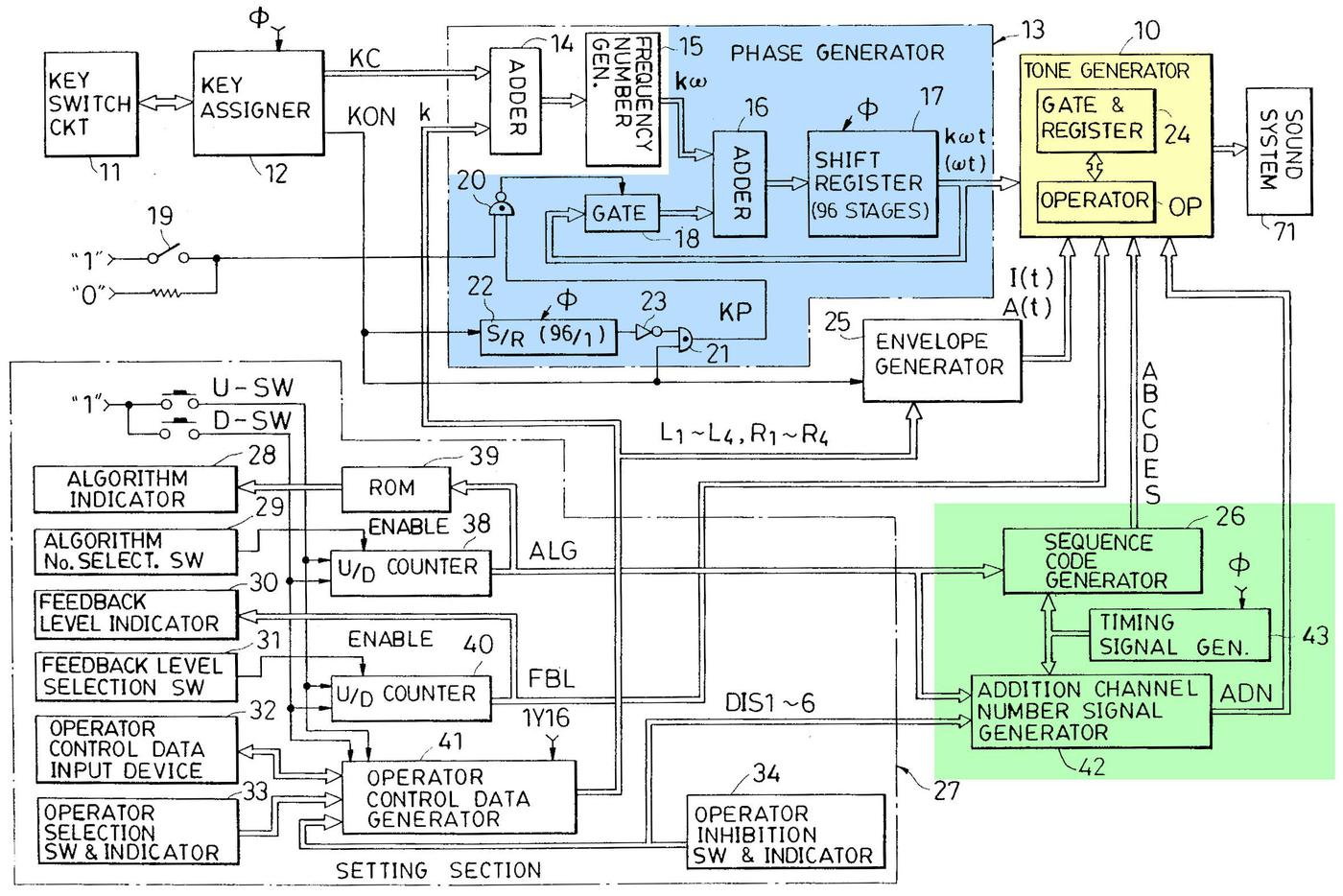Architecture diagram of the DX7, from the patent.

In more detail, the phase generator (blue) implements the phase counters for the 96 digital oscillators. The "frequency number generator" in the envelope chip provides the increment values to the adder. The phase values are stored in the 96-stage shift register. The tone generator (yellow) is where the modulation happens. It takes the phase values, modulates them, and converts them to sine waves, producing the output sound value. It also modifies the level of the signals, as specified by the envelope generator. The sequence code generator (green) generates control signals (A, B, C, D, E, S) that select how modulation takes place at each step. The implementation of these components will be described in more detail below.

### Logarithms and exponentials

The chip uses logarithms and exponentials for many of the internal values. The underlying problem is that multiplication is much harder to perform with hardware than addition, especially with 1980s-era technology. The solution is that the chip uses base-2 logarithms in many places because adding logarithms is equivalent to multiplying the values. (The chip uses lookup ROMs in combination with bit shifting to obtain the logarithms and exponentials.)

The first role for logarithms is in the frequency input to the chip: instead of a phase increment value, it receives the base-2 logarithm of the increment. The motivation is that note frequencies are related exponentially: for instance, going up one octave doubles the frequency. Thus, shifting a note requires multiplying the frequency. Since the envelope chip represents frequencies as logarithms, the multiplication becomes a quick addition. The envelope chip then passes the corresponding phase increment to the operator chip as a logarithmic value. The operator chip uses an exponential look-up ROM to convert this value back to a linear value.

The second role for logarithms is to apply the envelope that shapes the signal's amplitude. The envelope is a time-varying multiplicative scale factor, scaling the amplitude to, say, 70% or 30%. To avoid multiplication, the logarithm of the scale factor and the logarithm of the signal are added. A second exponential look-up ROM converts the result back to a linear value. The envelope is provided to the operator chip by the envelope chip in logarithmic form. The logarithm of the sine-wave signal is conveniently obtained by storing log2(sin(x)) in the waveform ROM instead of sin(x), so the logarithm is obtained "for free".15

## A look at the die

The diagram below labels the pins and the main functional blocks of the chip. The shift registers are the largest blocks of the chip, especially the phase shift registers in the upper left. ROMs are the second-largest blocks, especially the sine ROM and the two identical exponential ROMs. Adders provide most of the logic circuitry; there isn't much "random" logic compared to a processor chip, for instance. The chip has several bit shifters that shift a numeric value, multiplying or dividing it by a power of two.16 In this section, I look at the low-level circuitry of the die and how the functions are implemented.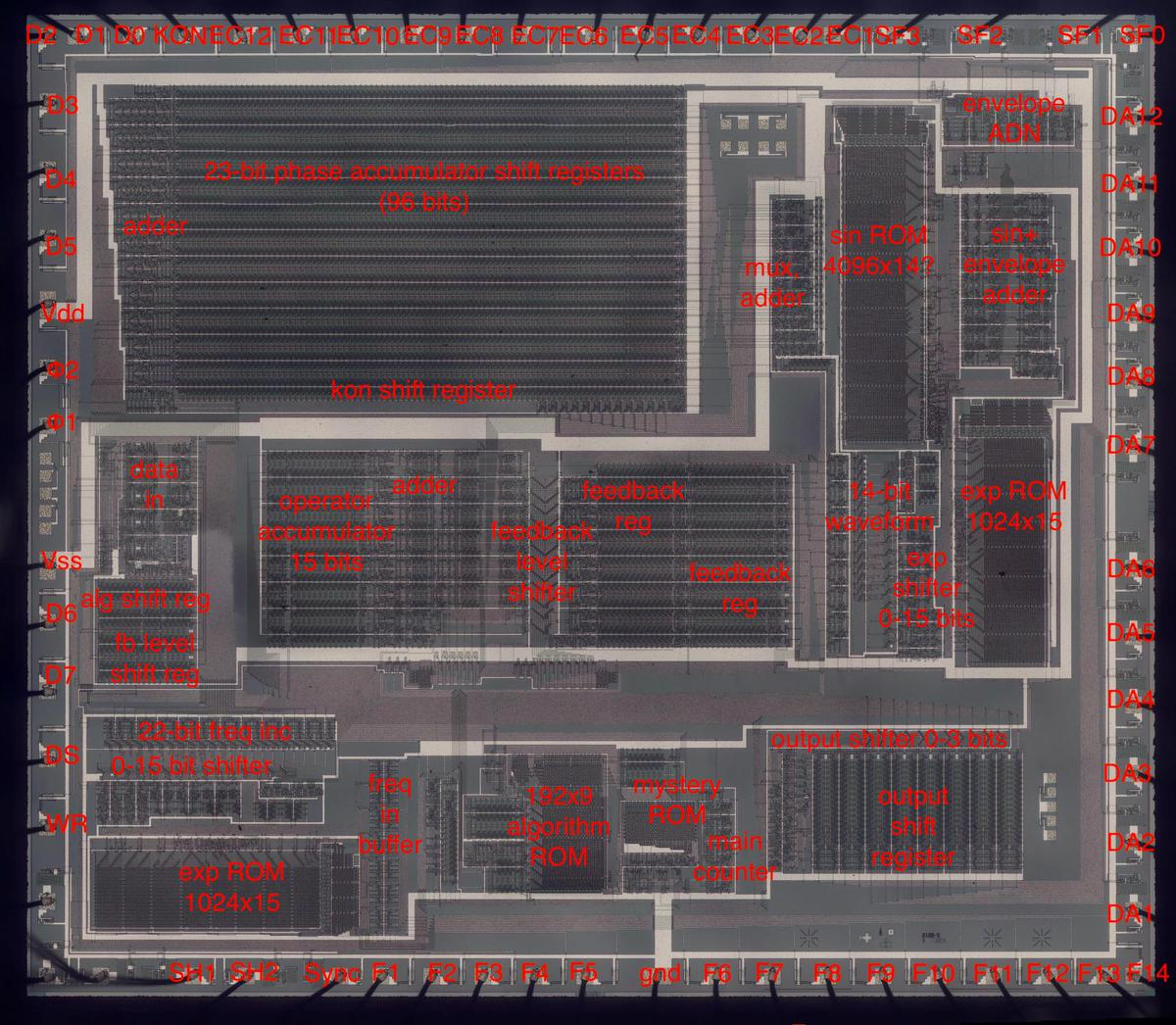Die with the pins and major functional blocks labeled. (Click for a larger version.)

### Shift registers

The main component of the chip is storage: the parameters for each operator, the phase counters for each oscillator, the output values for each note, and so forth. The storage is not implemented as RAM or fixed registers as you might expect, but as loops of shift registers with bits constantly moving in a cycle. The idea of a shift register is that it consists of a number of stages, say 16. During each clock cycle, the bits are shifted, with each bit moving to the next stage. One bit exits the shift register. This bit (or a new bit) can be fed into the shift register input, and it will appear at the output 16 clock cycles later.

Since the circuitry works on one oscillator/operator at a time in fixed order, shift registers are an efficient way of storing data and providing it at the right time, without the need for addressing logic. In other words, during each time interval, the appropriate data pops out of the shift registers for processing. The data (unmodified or modified as appropriate) is then fed back into the inputs of the shift register to pass through another cycle.

For example, each of the 16 notes requires 8 bits of configuration storage: 5 to specify the algorithm and 3 to specify the feedback level. This storage is implemented with 8 shift registers, each 16-bits long, as shown below. To select an algorithm, the external CPU writes the appropriate value into the shift register. Note that unlike RAM, entries in the shift register cannot be read and written arbitrarily. The system can only use values when they appear on the shift register output.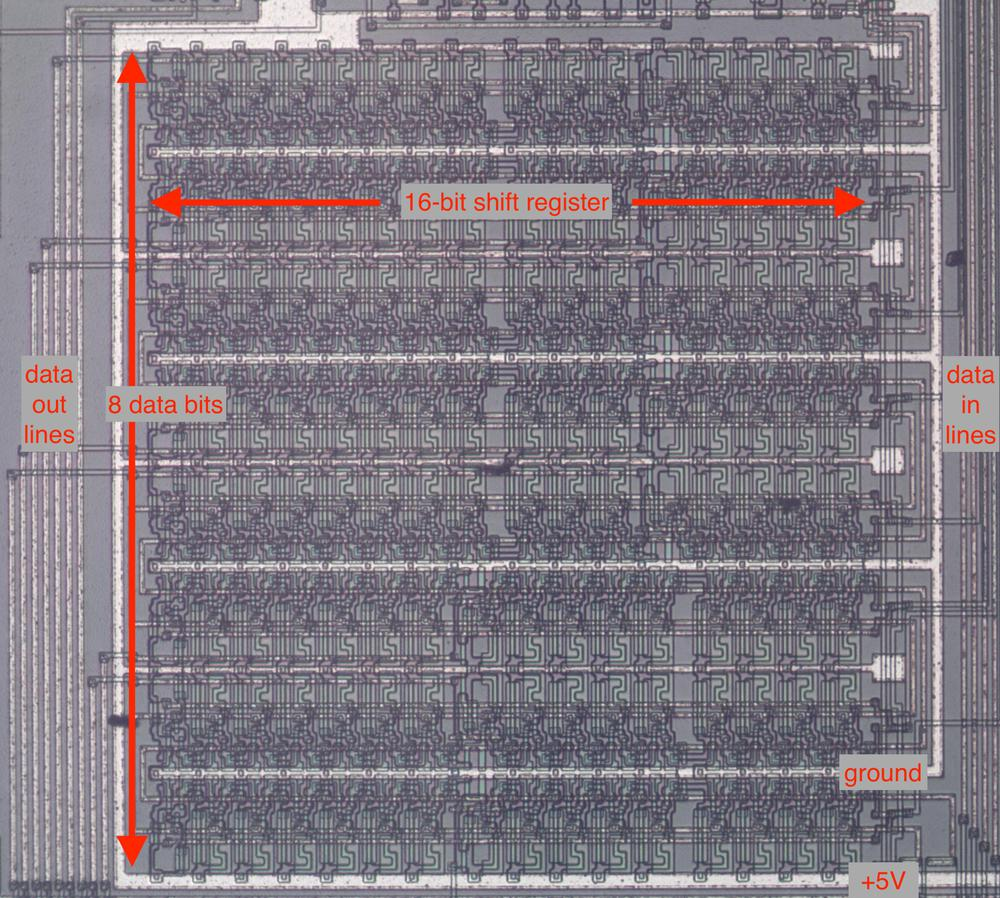The configuration data shift registers are organized as eight 16-bit shift registers.

The schematic below shows how one stage of the shift register is implemented. The chip uses a two-phase clock. In the first phase, clock ϕ1 goes high, turning on the first transistor. The input signal goes through the inverter, through the transistor, and the voltage is stored in the capacitor. In the second phase, clock ϕ2 goes high, turning on the second transistor. The value stored in the capacitor goes through the second inverter, through the second transistor, and to the output, where it enters the next shift register stage. Thus, in one clock cycle (ϕ1 and then ϕ2), the input bit is transferred to the output. (The circuit is similar to dynamic RAM in the sense that bits are stored in capacitors. The clock needs to cycle before the charge on the capacitor drains away and data is lost. The inverters amplify and regenerate the bit at each stage.)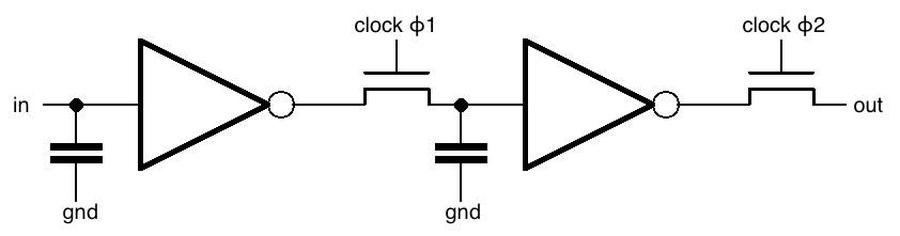Schematic of one stage of the shift register.

The diagram below shows the physical implementation of one shift register stage. It's a bit confusing because there are three layers: the whitish metal on top, doped silicon regions on the bottom (which appear outlined in black), and polysilicon lines in the middle (which appear reddish or greenish). Transistors are formed when a polysilicon line crosses doped silicon. A capacitor is created similarly, with a polysilicon line and doped silicon forming the two plates of the capacitor. An inverter is created from a transistor that pulls the output to ground, along with a pull-up resistor. (The pull-up resistor is actually another transistor, specially doped to make it a depletion transistor.)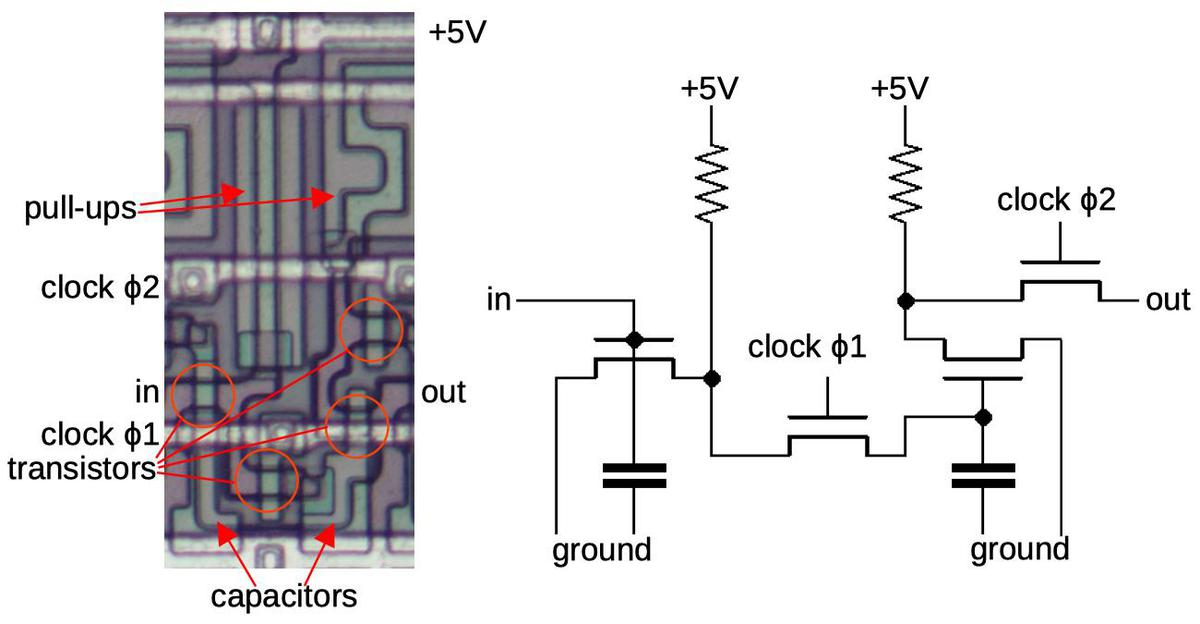Implementation of one bit of the shift register. This matches the earlier schematic, but shows the components of the inverters.

### ROMs

The next building block of the chip is ROM storage, used for the numeric look-up tables and other purposes. One ROM computes the log2 sine for the waveform. The chip has two identical exponential ROMs computing 2x. One converts the log-frequency increment value into a linear increment value. The second converts the log waveform value into a linear waveform value. An algorithm ROM defines the 32 algorithms, specifying the behavior of each of the 6 operators in each algorithm. Another ROM changes the behavior of different notes and operators in a way that is still a mystery to me.

A ROM is arranged in a grid. At each position, silicon is doped to either create a transistor or no transistor, representing a 0 or 1. In a typical ROM, five address bits energize one of 32 vertical select lines to select one column of the ROM. The rows are organized in groups of 8 and three more address bits select one row from each group to yield output bits.

The diagram below shows part of the ROM circuitry. The magnified portion has been colored to show the bits. The vertical column select lines of polysilicon are colored yellow. The ROM is programmed by the pattern of doped silicon (blue). A transistor (red) is formed when a polysilicon line crosses a doped silicon region; the transistors are indicated in red and indicate the bit pattern.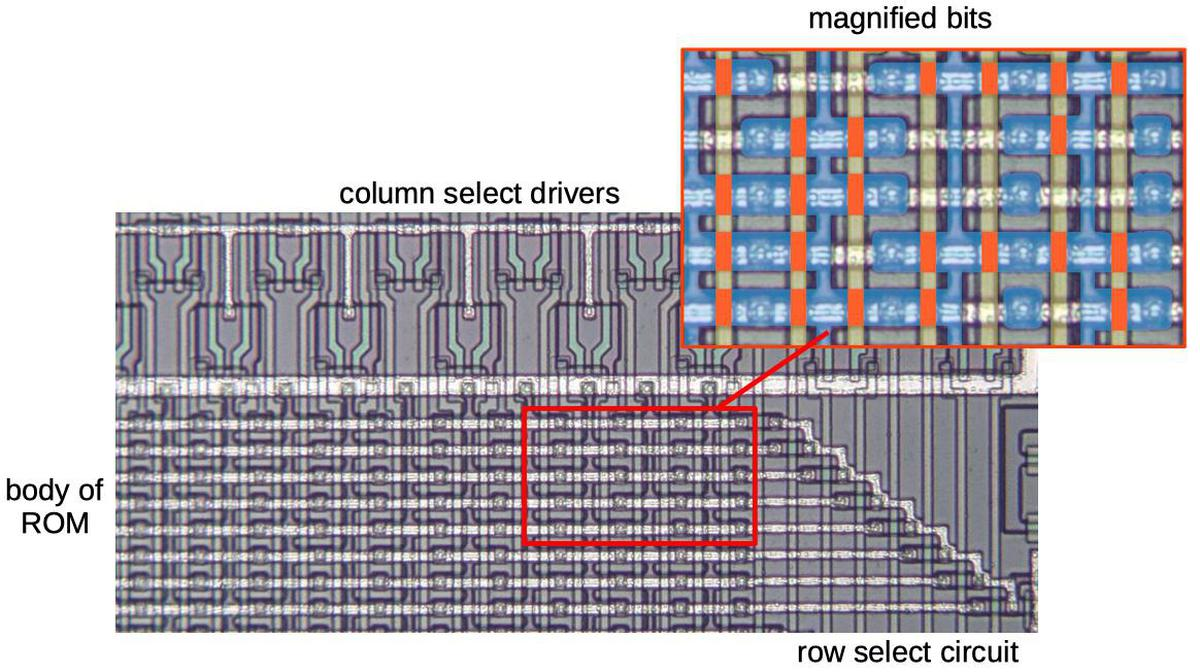Closeup of the log-sine ROM showing individual bits.

The ROMs use several tricks to reduce space. Duplicate rows are folded together, such as high-order bits that are zero for a range of values. The sine ROM apparently uses delta encoding for alternating values; since the delta values are small, they have a lot of zero bits that can be folded. As a result, the values stored in the ROM are not obvious from the bit patterns. I'm still investigating the ROM representations and will discuss them later.

Another key building block of the chip is the adder, which sums two binary numbers. The chip has multiple adders: for the phase accumulators, inside the operators, and to apply the envelope.

A multi-bit adder is built from full adders, a circuit that adds two bits (along with a carry-in bit), and produces a sum bit (along with a carry-out bit). The diagram below shows how a one-bit full adder is implemented, adding bits A and B along with a carry-in, producing an output sum bit and a carry bit.17 Note that the outputs are inverted; other parts of the circuitry deal with that.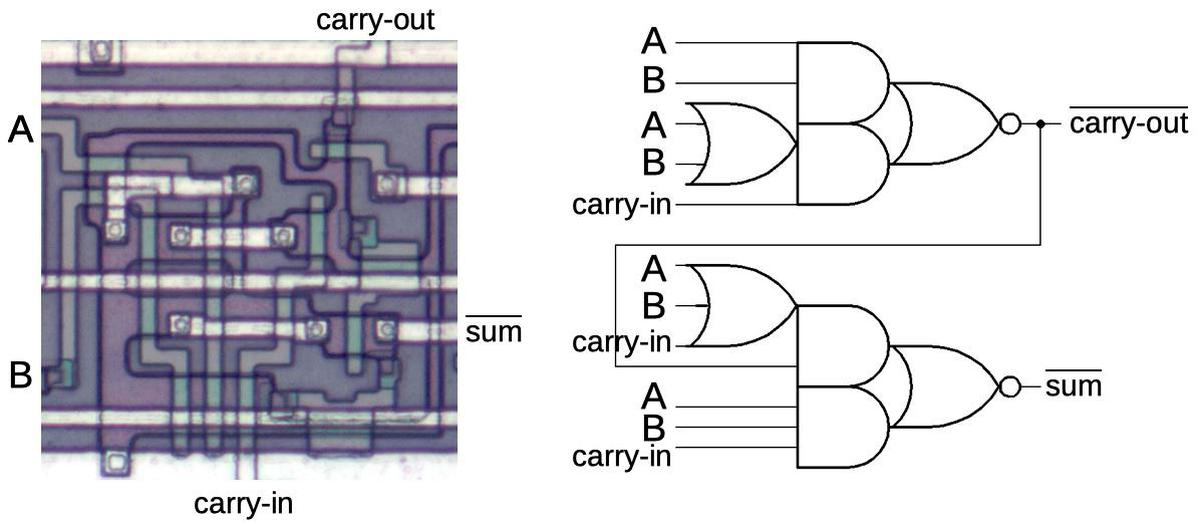Structure of the full-adder circuit used in the chip.

By combining multiple one-bit adders, multi-bit binary numbers can be added as shown in the 23-bit adder below. Note that the adder is at an angle relative to the shift registers. This is a clever trick for performance. One problem with adders is dealing with carries, which may need to propagate through all the bits. (The binary equivalent of needing to repeatedly carry the 1 when computing 999999+1.) The solution is to break the sum into 6 parts. Only 4 bits of each sum are added in each clock phase, so the carry only needs to propagate through 4 bits rather than all 23. The next chunk is added in the next clock phase, and so on.18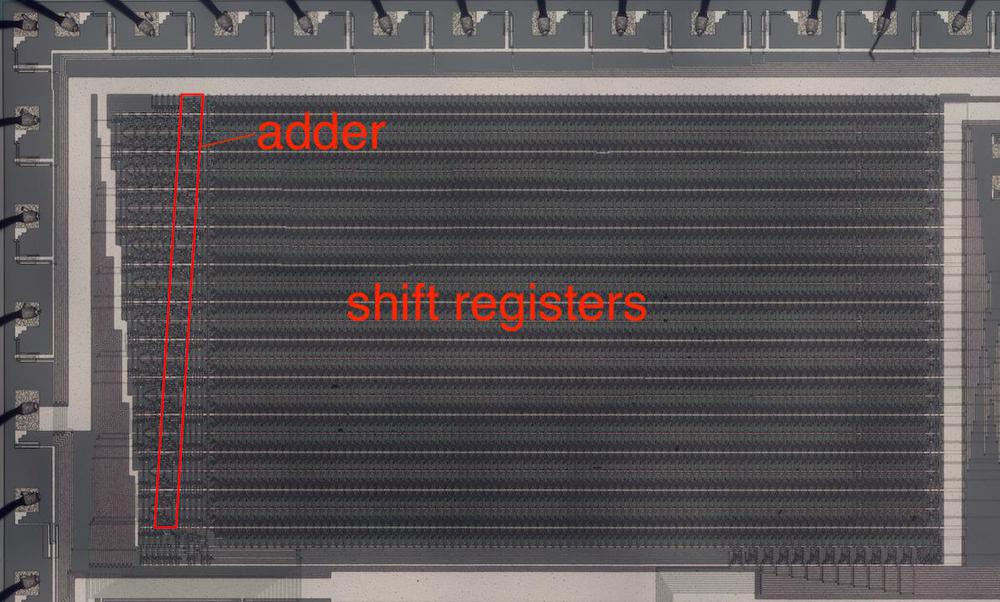The phase adder is at the left of the shift registers that hold the 96 phase values.

### Bit shifter

The final building block that I'll discuss is the bit shifter, which shifts a binary value left or right numerically, which is equivalent to multiplying or dividing by a power of 2. A typical shifter is built in two layers: the first layer shifts by 0, 1, 2, or 3 positions. The second layer shifts by 0, 4, 8, or 12 positions. The combination of the two layers permits any shift between 0 and 15 bit positions.

The diagram below shows part of the shifter that shifts by 0, 1, 2, or 3 positions, controlled by the horizontal lines. I've highlighted one of the bits in green. If the "shift 0" line is activated, the leftmost green transistor (circled) will turn on and the green input bit will exit unshifted at the first output position. Likewise, if the "shift 1" line is activated, the second green transistor will turn on and the green bit will exit at the second position, shifted one position to the right. The "shift 2" and "shift 3" lines will cause the green bit to exit two or three positions to the right. The remaining transistors (circled in black) act in the same manner to shift the other bits. The result is that all the bits will pass straight throw (shift 0), or be shifted 1, 2, or 3 positions to the right.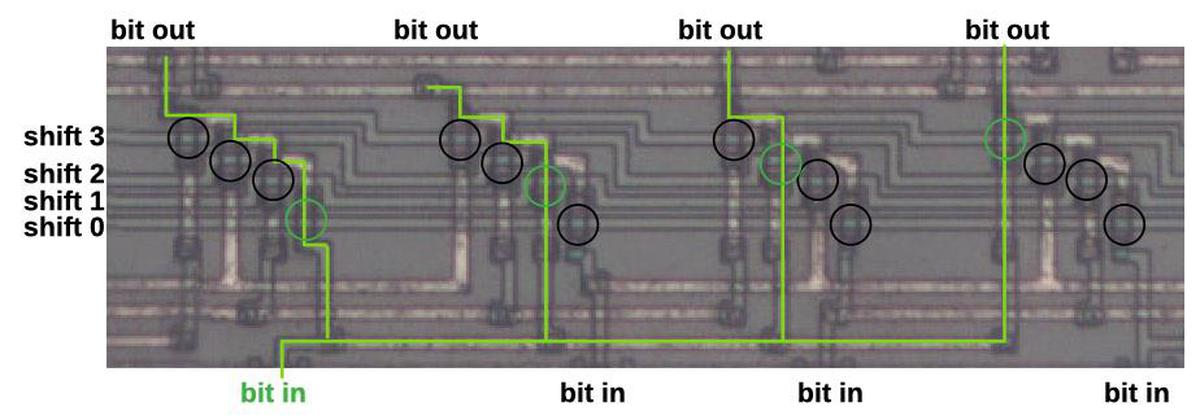Detail of a shifter circuit.

Shifters are used in combination with the exponential ROMs to compute 2x. The ROM is applied to the fractional part of x, while the shifter is controlled by the integer part. This is much more efficient than using a large ROM to look up the complete value. Another shifter provides a shift of 0 to 6 bits to scale the operator feedback value. A shifter also scales the output value to increase the dynamic range.

## Combining and modulating operators with an algorithm

The DX7 generates each note by combining and modulating six operators (oscillators) according to a particular algorithm. This happens sequentially: the chip processes operator 6 for channels 1 through 16, then operator 5 for all the channels, and so forth, ending with operator 1. This cycle of 96 operations repeats, providing new sound values 49096 times a second.19

The diagram below shows a typical algorithm. Operator 6 modulates operators 4 and 5, while operator 3 modulates operators 1 and 2, as well as itself. Operators 1, 2, 4, and 5 produce outputs, which are combined to create the final sound value. This section discusses the circuitry that performs the modulations for the specified algorithm.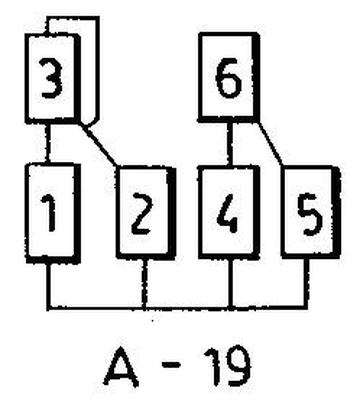Algorithm #19 combines the 6 operators in a specific way.

The diagram below shows the implementation of the circuitry to process operators. The lower "operator" box is the circuitry previously discussed: the first adder adds the modulation value f(ωmt) to the current phase value kωt and looks up the value in the sine table. The second and third adders apply the envelope. Finally, the log/linear converter is implemented by the exponential ROM and shifter described earlier.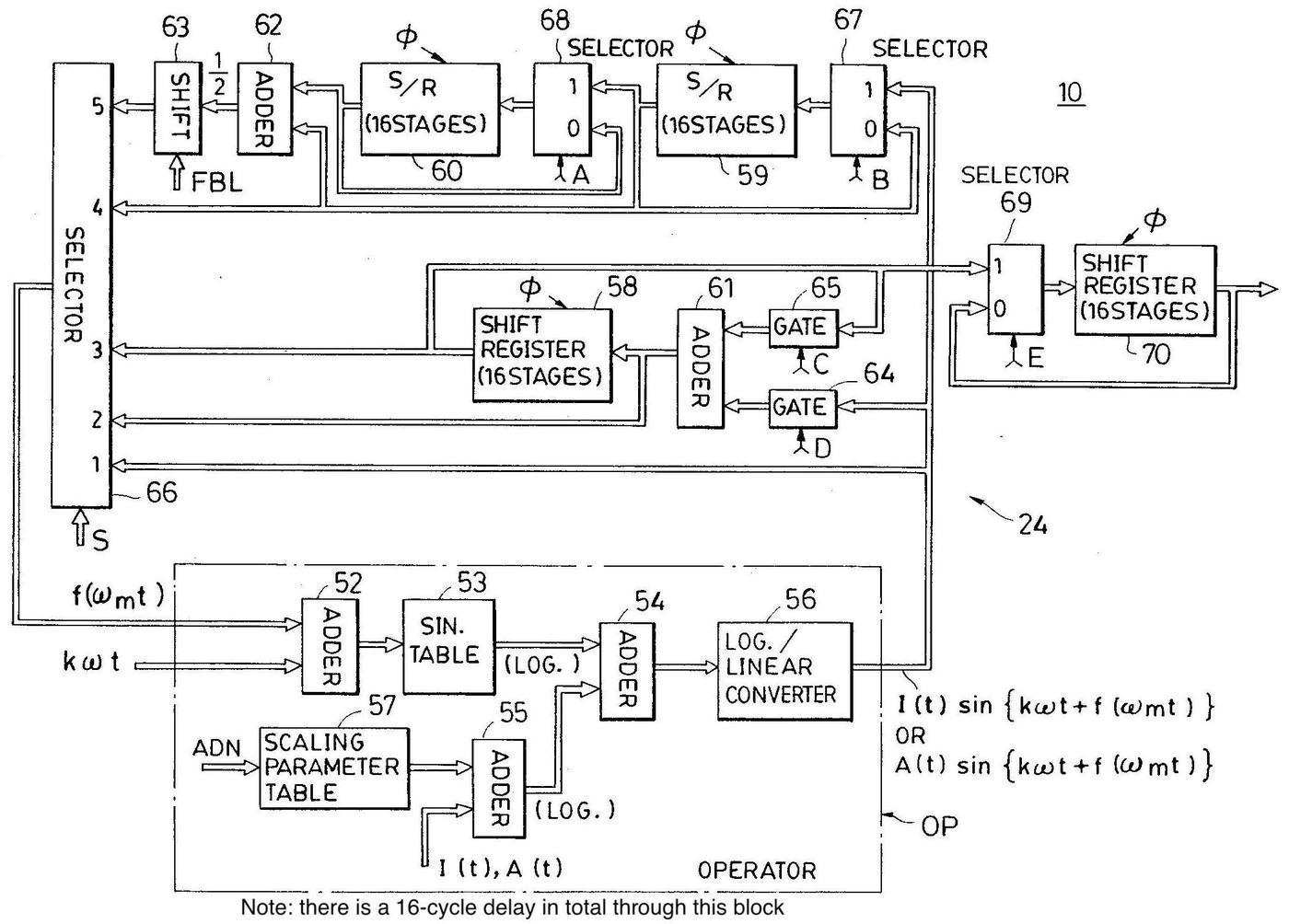Diagram showing the construction of an operator, from the patent.

The upper half of the diagram determines the appropriate modulation value f(ωmt) for the selected algorithm and operator. This circuitry is complicated, since there are 5 different cases that the circuitry must handle, chosen by the selector.20 The top circuit (selector input 5) implements the feedback of an operator to itself. To provide feedback, the previous two values are stored in 16-stage shift registers, scaled by the feedback level parameter (FBL), and output as the modulation value. (Two previous values are averaged to stabilize the feedback.) Since the 16 channels are processed in sequence, the 16-stage shift registers store the feedback values until the next cycle. The next circuit (selector 4) uses the value of the self-feedback operator to modulate another operator. Selector 3 provides a shift register and adder to sum or delay values. (It is where multiple values are summed to produce the final output.) Selector 2 allows a sum to be used for modulation. Selector 1 is the simple case where the previous operator provides the modulation (e.g. 6 modulating 5). Finally, if no value is selected, the signal remains unmodulated. Control signals A, B, C, D, and E select the specific signal paths.

The diagram below shows the implementation of the modulation circuitry on the die. This circuitry corresponds to the upper part of the patent diagram above; the component numbers match the patent numbers. This circuitry occupies the middle portion of the die, with the shift registers taking up the bulk of the space. The adders and feedback level shifter are also visible.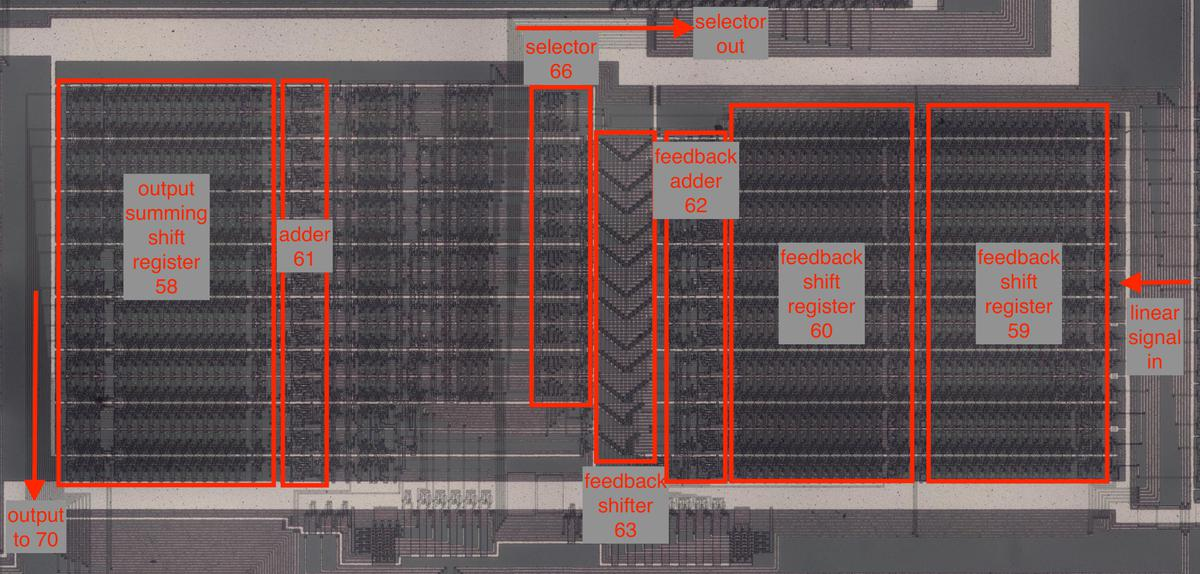Implementation of the modulation circuitry on the die.

The algorithms are specified by the algorithm ROM (below). This 192×9 ROM produces 9 control signals for the 6 operators in the 32 algorithms. The 16-stage shift register described earlier holds the selected algorithm numbers and provides the input to the ROM. Curiously, it appears that the chip permits each of the 16 notes to use a different algorithm, even though the DX7 does not support this feature.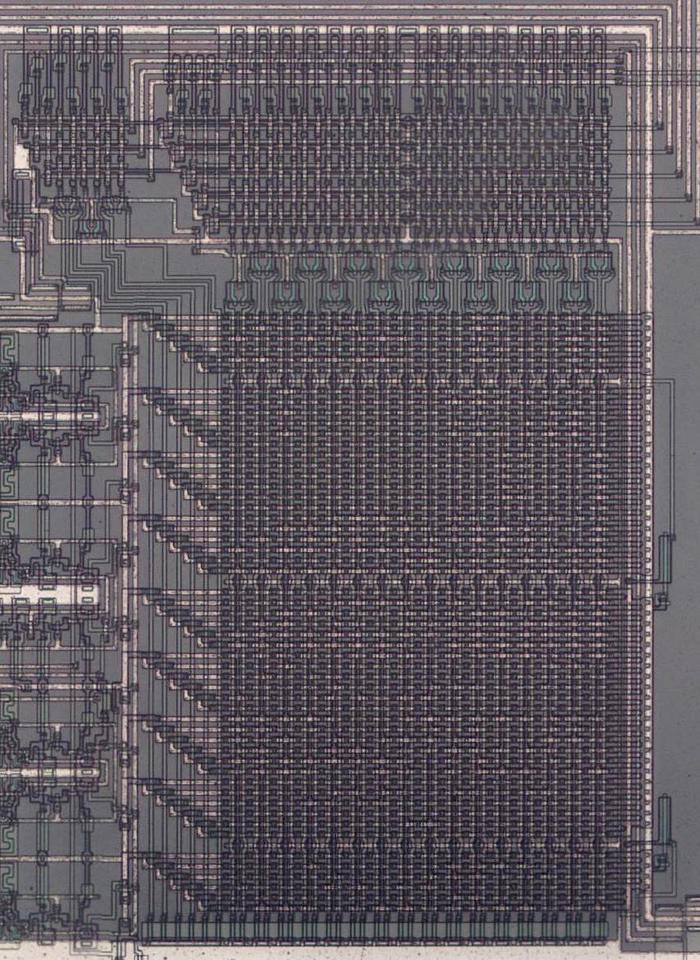The algorithm ROM. The circuitry at the top decodes the address (algorithm and operator number), selecting a column from the body of the ROM below. The 9 outputs (A, B, C, D, E, and S) are at the left.

## Conclusion

The DX7 was a groundbreaking synthesizer and this chip was at the heart of it, so in a sense this chip was responsible for the 80's sound. Studying the chip's die reveals some interesting circuits. Uncovering the secrets of how the chip operates may help build more accurate DX7 emulators. The chip is complex and this article just scratches the surface so I plan to study the chip in more detail. In particular, I intend to extract the data from the ROMs to find out exactly how the waveforms are represented. In any case, I hope you've found this deep dive into a sound chip interesting.

I announce my latest blog posts on Twitter, so follow me @kenshirriff. I also have an RSS feed. Thanks to Jacques Mattheij and Anthony Richardson for providing the chip and discussion.

## Notes and references

1. The Economist published an article on how the DX7 changed modern music. The article called the DX7 "one of the most important advances in the history of modern popular music," altering the soundscape more than any instrument since the electric guitar.

2. The 40% number is from Prof. Megan Lavengood's detailed research on the DX7, in particular What Makes It Sound '80s: The Yamaha DX7 Electric Piano Sound. One interesting factor from Lavengood's research is the importance of preset sounds in the DX7, a feature that most earlier synthesizers didn't have. As a result, most users didn't program the DX7 but just pressed a button to use a preset sound. Programming the DX7 was much more difficult than analog synthesizers both because of the non-intuitive nature of FM synthesis and the DX7's arcane user interface: buttons and menus rather than knobs and sliders that provided immediate feedback. The DX7 also "democratized" the use of synthesizers through its low price: under \$2000 (at the time), much cheaper than competing synthesizers. (The Fairlight CMI was \$25,000 in comparison.)

3. To hear the DX7's 32 classic factory patches, check out this video. Some good examples of 80s songs using these patches are in this video

4. The DX7 contains two CPUs: a Hitachi 63B03 and a Hitachi 6805S, both related to the 8-bit Motorola 6800. These processors manage the keyboard, user interface controls, MIDI communication, low-frequency oscillator, and so forth. These processors were not powerful enough to do the sound synthesis; they sent data to the envelope and synthesis chips, which generated the sounds.

5. It's unclear if the official part numbers of the chips are YM2128/YM2129 or YM21280/YM21290. The chip package and die are labeled YM2128, but the circuit board, schematic, and documentation are labeled YM21280. The chip is also known as the FM Operator Type S chip or OPS chip.

6. I estimate that the chip has about 45,000 transistors, a bit less than the 80186 processor (1982). I measure the feature size as 3 µm, a step behind the 1.5 µm process introduced in 1981. My conclusion is that the chip was advanced, but not quite cutting-edge. The die is approximately 7.6×6.6mm.

7. The photo below shows the YM21280 chip, showing the staggered pins.The Yamaha YM21280 chip. Photo courtesy of Jacques Mattheij.

8. I'm going over synthesizer history extremely briefly, so I'm oversimplifying things. For instance, there are different architectures for analog synthesizers, multiphonic analog synthesizers, digitally-controlled analog synthesizers, and so forth. Wikipedia provides a detailed history.

9. Typically, an envelope generator used an ADSR (attack, decay, sustain, release) model. The attack is the spike in amplitude when the key is pressed, followed by a decay to a lower level. The note remained at the sustain level as long as the key was pressed, and then fell off during the release level. The times and levels could be adjusted as desired. For example, a piano-like sound has a rapid attack and decay for the initial sound, while a trumpet-like sound would have a slower attack as the note builds.

10. The Musical Instrument Digital Interface (MIDI) standard was announced in 1982, allowing synthesizers to be controlled over a digital link. MIDI could be used for remote keyboards, playing notes via a sequencer, computer composition, and other applications. Although MIDI is a digital protocol, the first synthesizers to use it were analog, such as the Roland Jupiter-6, converting the digital messages to analog control voltages.

11. Technically, the DX7 uses phase modulation (PM) instead of frequency modulation (FM), but the two techniques are related. In phase modulation, the basic frequency stays constant but the phase of the signal is increased or decreased. But if the phase increases, the oscillations happen faster so the frequency is increased. Likewise, a decrease in phase stretches out the waveform, reducing the frequency. It turns out that phase modulation is the same as frequency modulation using the derivative of the modulation signal. (Note that if the phase shift is constant, the PM output has the original frequency, just shifted in time. But a constant modulation signal for FM results in a constant frequency shift.)

Since the derivative of a sinusoid is another sinusoid, an FM signal and a PM signal look the same with sinusoidal modulation. However, the derivative is scaled by the frequency, with the result that PM signals are more sensitive to modulation by high frequencies than low frequencies. (An FM signal will have the same frequency sweep with slow modulation and fast modulation, while a PM signal will have little frequency change if the modulation is slow.) The results of frequency modulation and phase modulation will also be different for non-sinusoidal modulation, since the derivative will be different from the modulation signal.

12. Note that the frequency resolution in this example isn't very good if you use integers for the increment size. For example, an increment of 44 gives 440 Hz and an increment of 45 gives 450 Hz and you can't get a frequency in between. The solution is to include a fractional part in the increment and index to provide more control.

13. My synthesis widget illustrates FM synthesis (actually PM synthesis) in general. It doesn't simulate the DX7 specifically.

14. The DX7's envelopes are complex. A typical synthesizer's attack-decay-sustain-release envelope is defined by four parameters: the attack speed, decay speed, sustain level, and release speed. The DX7's envelope has eight parameters: L1-L4 and R1-R4, defining both the level and rate for the four phases, providing more control. Each of a sound's 6 operators has its own envelope, adding even more complexity.

15. I don't know yet how the negative half of the sine wave is represented logarithmically. My guess is that the sign is represented separately so the waveform remains positive.

16. Note that the bit shifters are unrelated to the shift registers, both in design and function. The shift registers shift are used for storage, shifting numbers through time. The bit shifters operate numerically, scaling a number.

17. The adder's complex gates make more sense if you think through the cases. You'll have a carry-out if both inputs A and B are set. You'll also have a carry-out if you have a carry-in and at least one of A or B. The sum bit will be set if you have A, B, and carry-in set, which is handled by the lowest AND gate. The sum bit will also be set if you have at least one of A, B, and carry-in, but you need to exclude the case where two of them are set, which is handled by ANDing in the inverted carry-out.

The underlying reason for the complex OR-AND-NOR logic instead of multiple, simpler gates is that each NMOS gate requires a pull-up resistor. Thus, one complex gate may be smaller than several simple gates because you reduce the number of pull-up resistors.

18. The adder can be viewed as a six-stage pipeline, with each stage adding a few of the bits. A sum needs to pass through all the stages to be completely added. Note that the stages are all active at the same time, but they are acting on different sums.

19. Note that the algorithms are carefully designed so operators are modulated only by operators with a higher number. Thus, starting at #6 and ending at #1 ensures that values are calculated in the right order. The 32 algorithms make it look like almost anything is possible, but the hardware creates several constraints that limit the possibilities. For instance, there is only one sum/delay register so you can't sum modulators and the output at the same time. You can't delay a non-feedback operator after an output takes place; for instance, algorithm 11 has 6 delayed to modulate 3, but only because there haven't been any outputs at that point. You can only have one self-feedback loop.

20. The operator circuit is a bit tricky to understand. One factor to keep in mind is that the computation is spread out over time, computing one operator at a time. Moreover, the computations are interleaved across the 15 voices, so data needs to be stored in a shift register until the next operator is processed. Although the algorithms look straightforward in the diagrams ("operator 6 feeds into operator 5"), the implementation becomes complicated when this is split into time slices.

21. Patent 4554857 "Electronic musical instrument capable of varying a tone synthesis operation algorithm" provides detailed information on the architecture of the DX7 synthesizer. The DX7 Schematics provide circuit-level information, including the chip pinout (below). The DX7 Technical Analysis page summarizes what is known about the DX7's internals.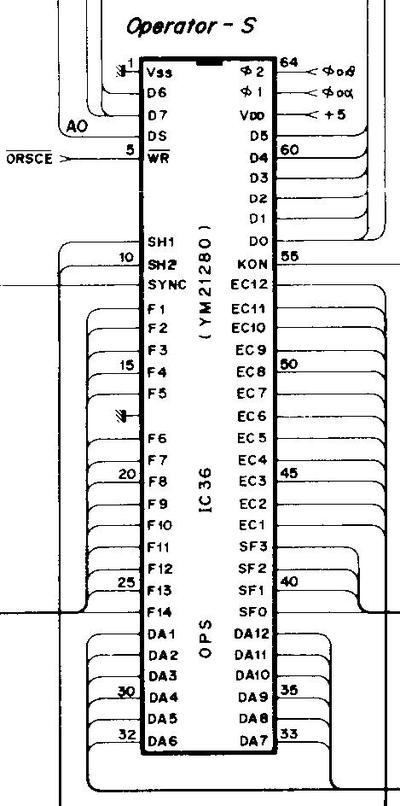The DX7 schematic provides the chip's pinout.